# RS Aggarwal Solutions for Class 7 Maths Chapter 20 Mensuration

RS Aggarwal Solutions for Class 7 Maths Chapter 20 – Mensuration are provided here. While solving the exercise questions from the RS Aggarwal book, students often face difficulty and eventually pile up their doubts. Our expert tutors formulate these exercises to assist you with your exam preparation to attain good marks in Maths. Students who wish to score good marks in Maths practise RS Aggarwal Class 7 Solutions

These Mensuration solutions are available in RS Aggarwal Solutions for download in pdf format. They provide solutions to all the questions provided in Class 7 Maths Chapter 20 wherein problems are solved step by step with detailed explanations. Download pdf of Class 7 Chapter 20 in their respective links.

## Download PDF of RS Aggarwal Solutions for Class 7 Maths Chapter 20 – Mensuration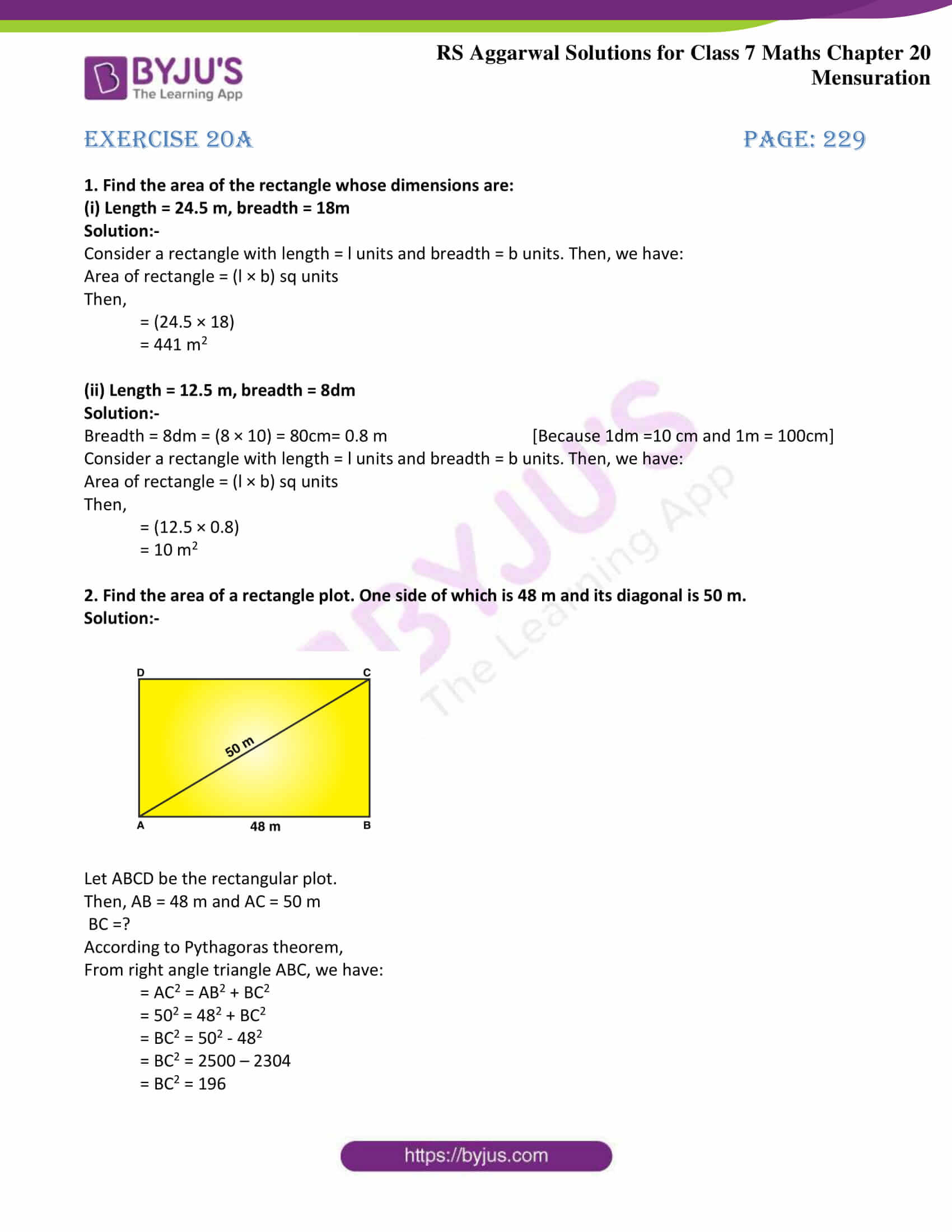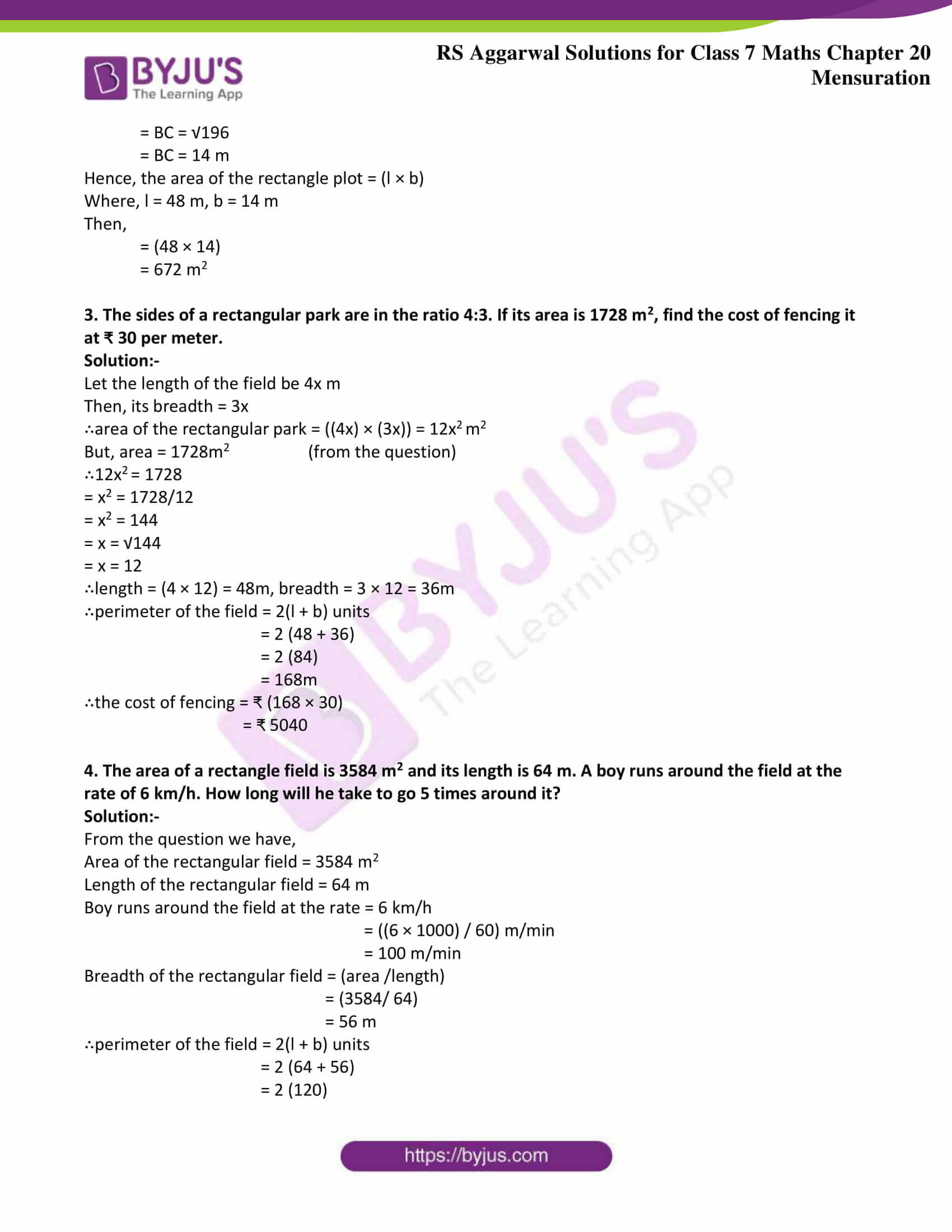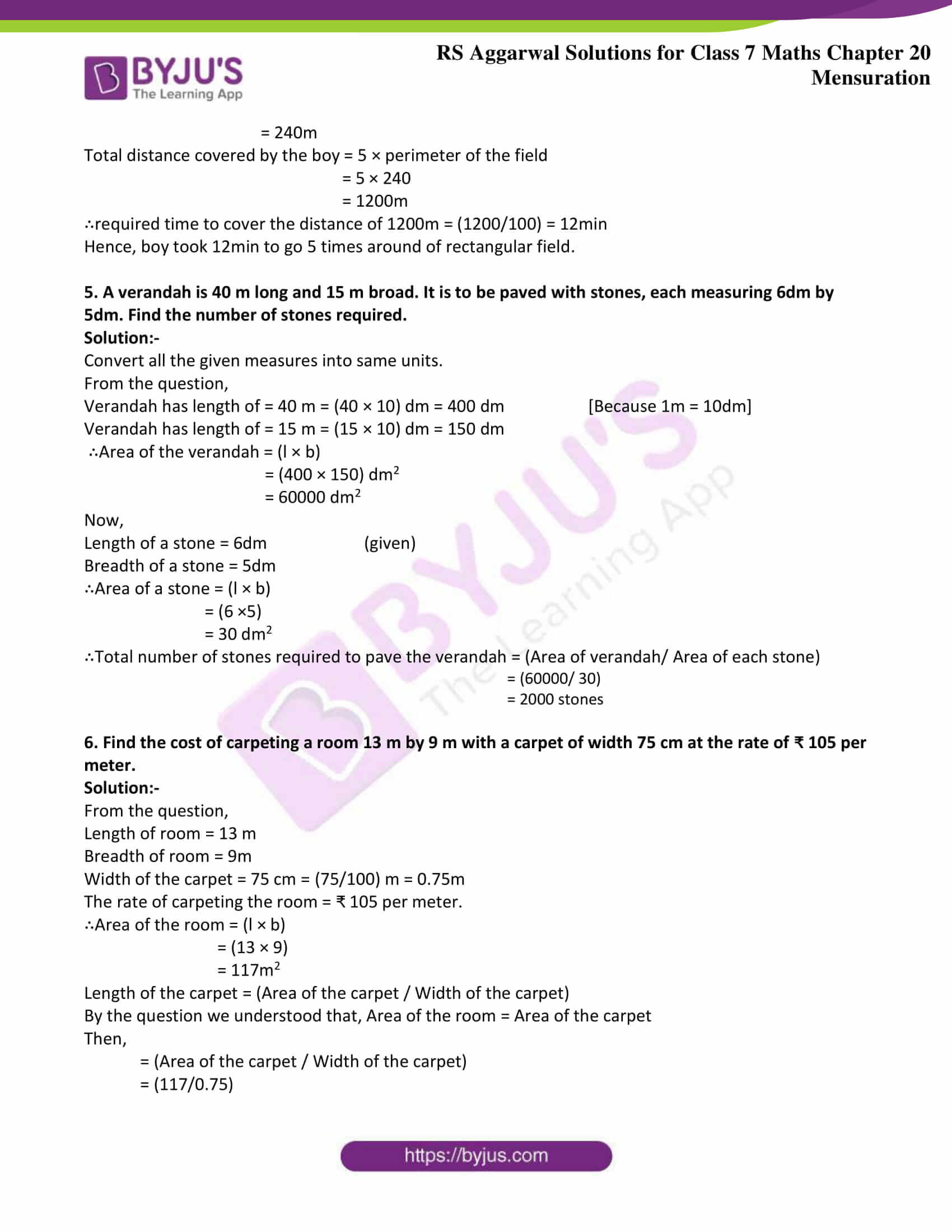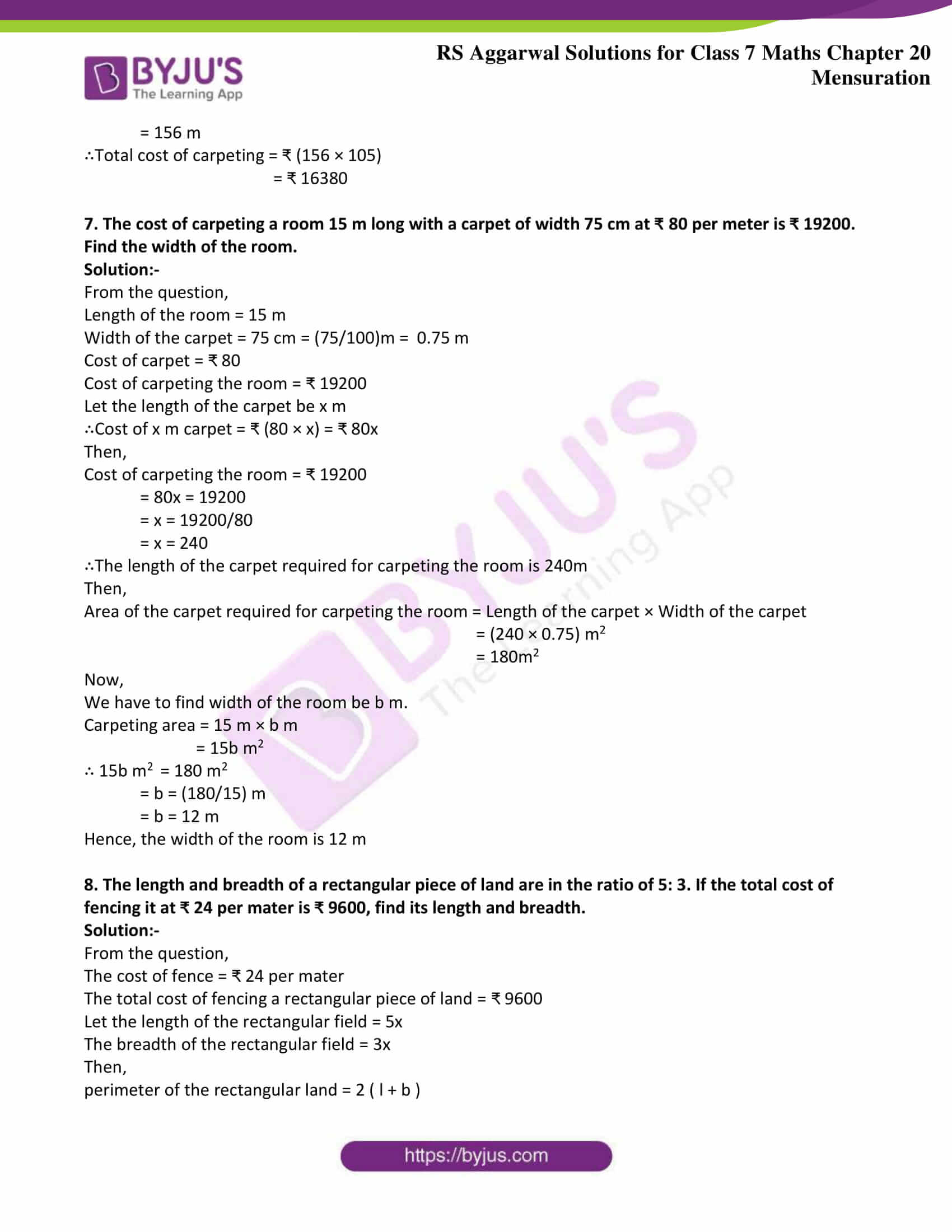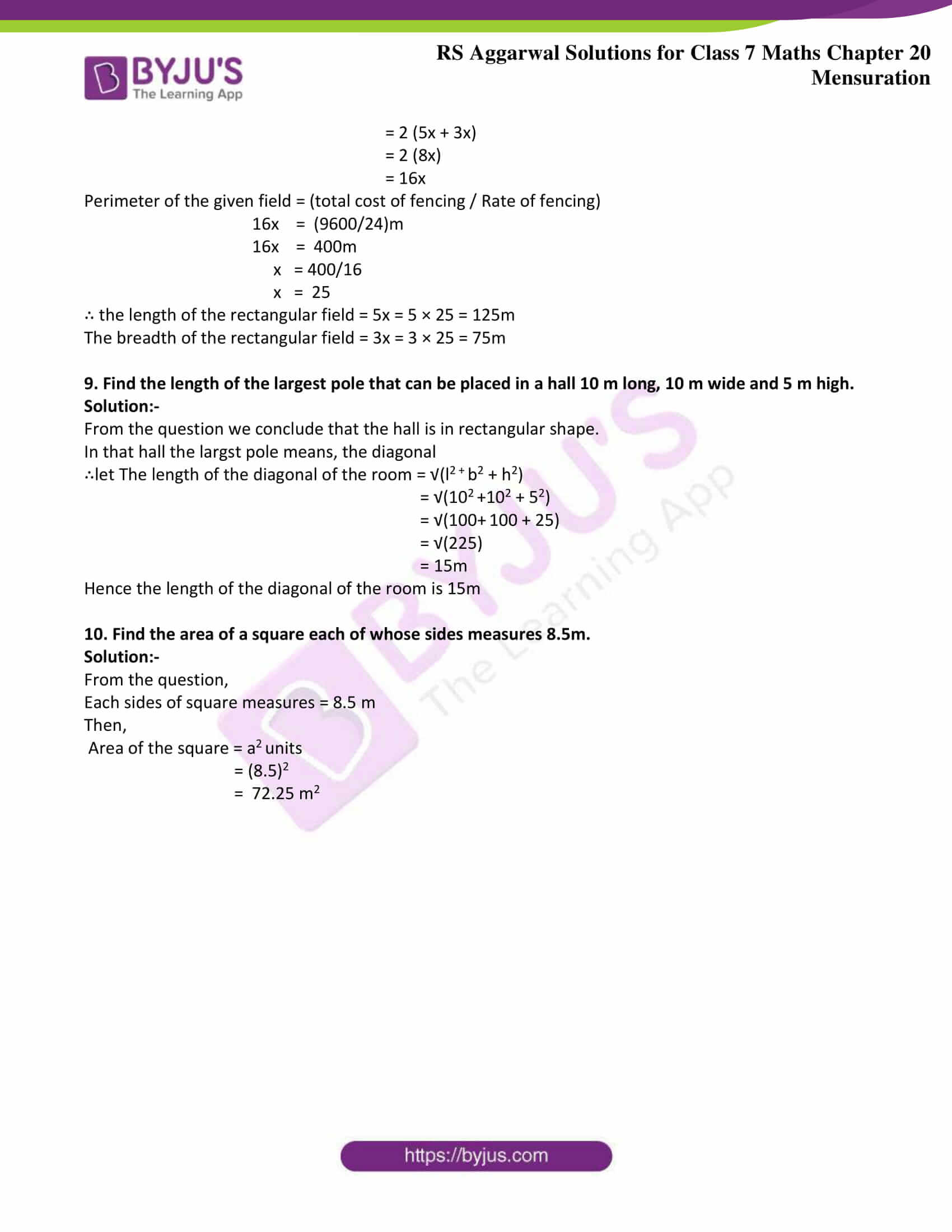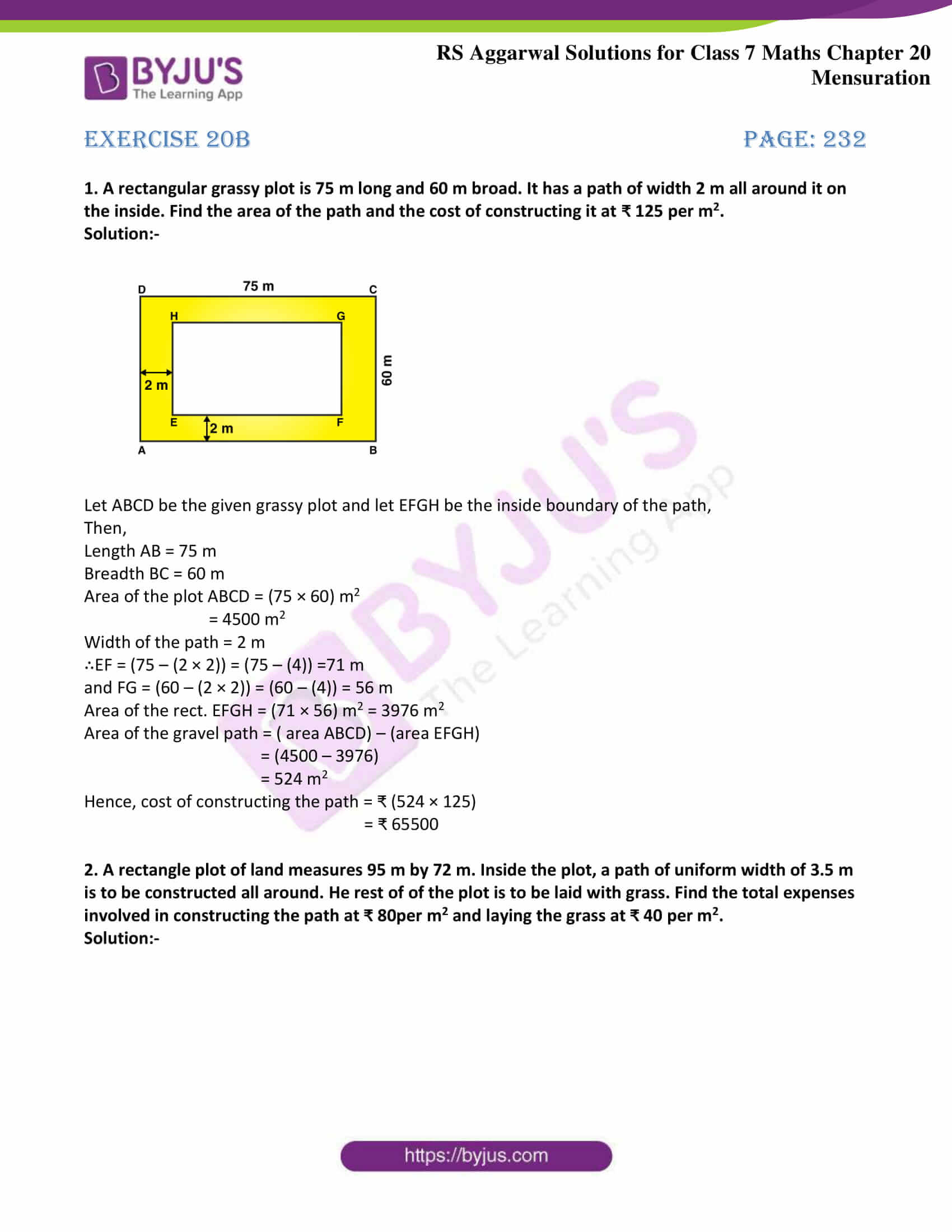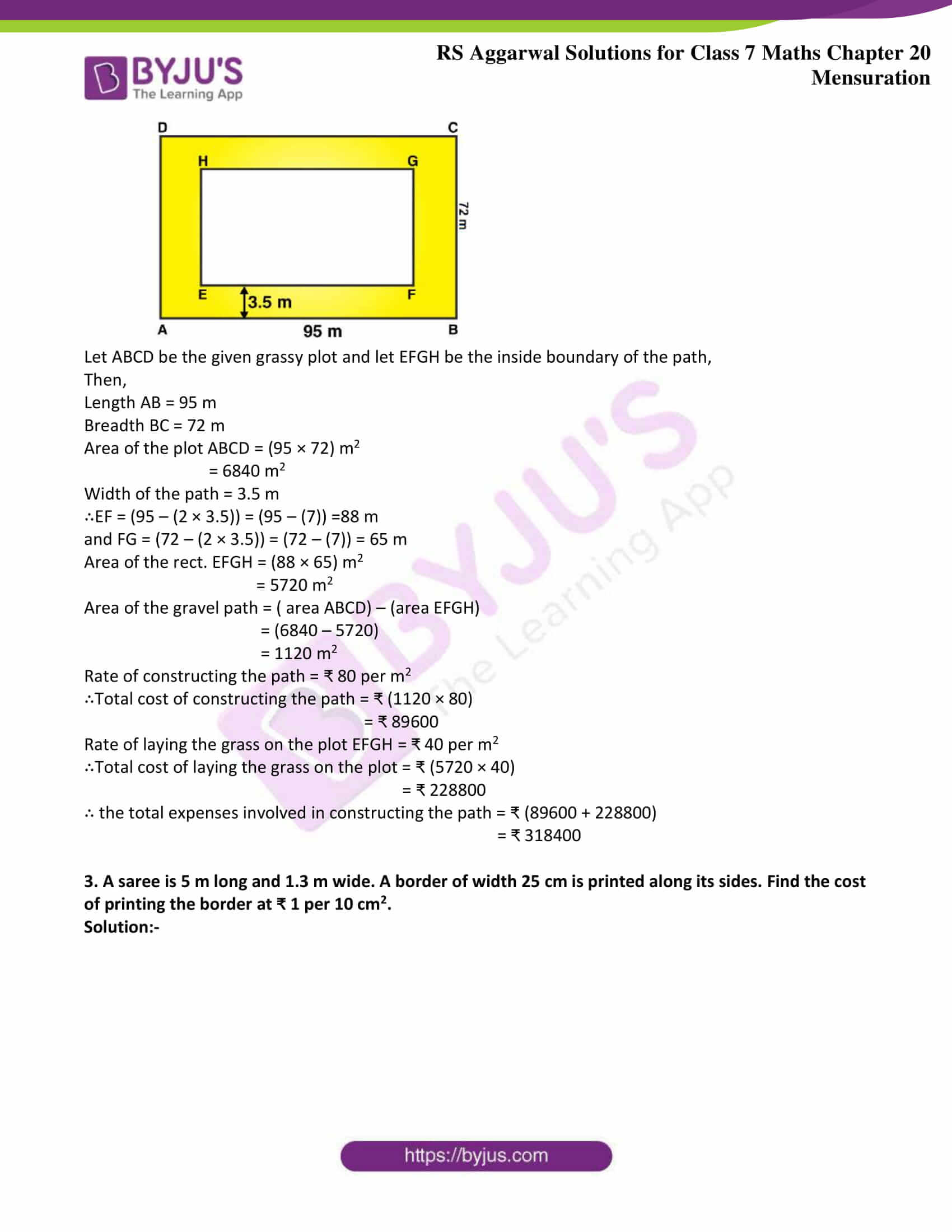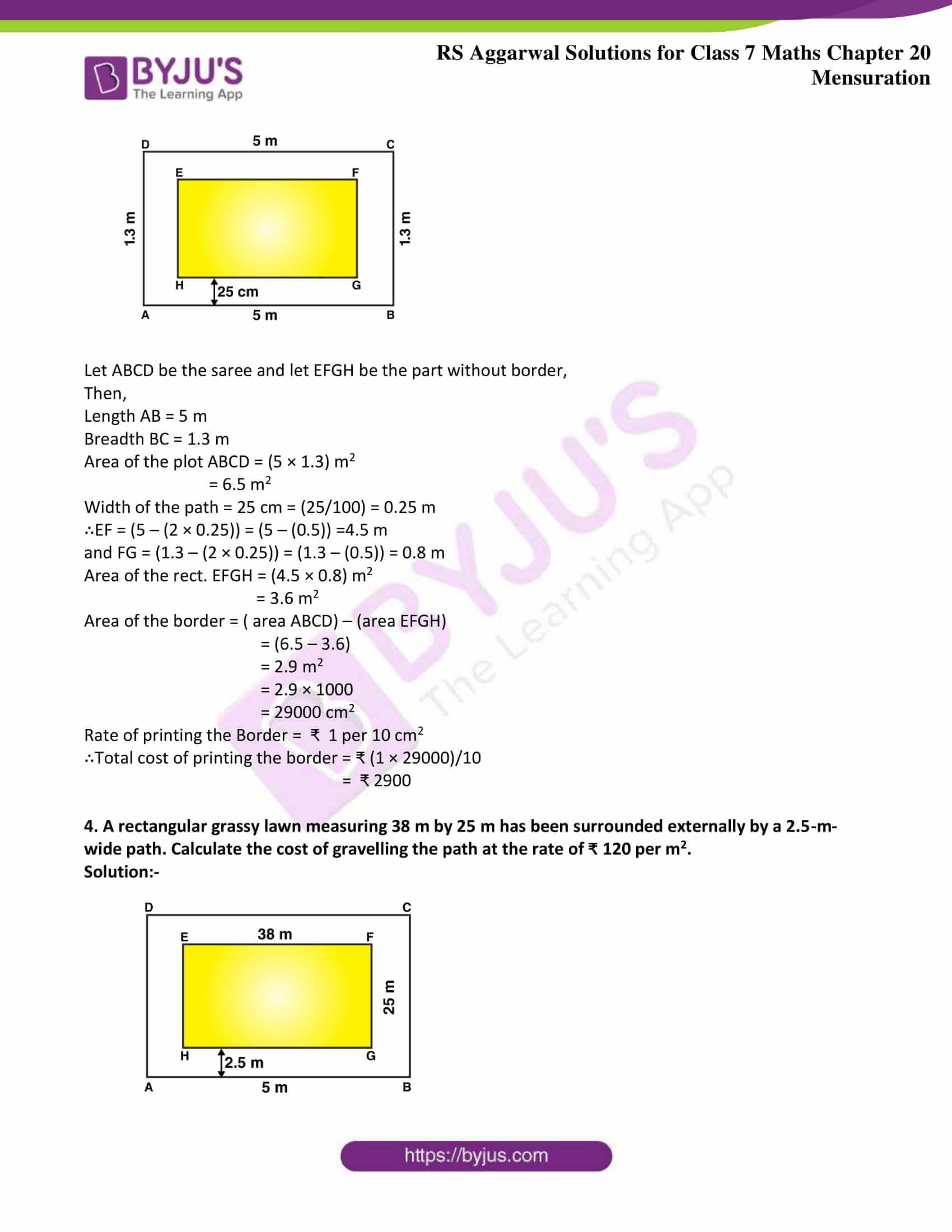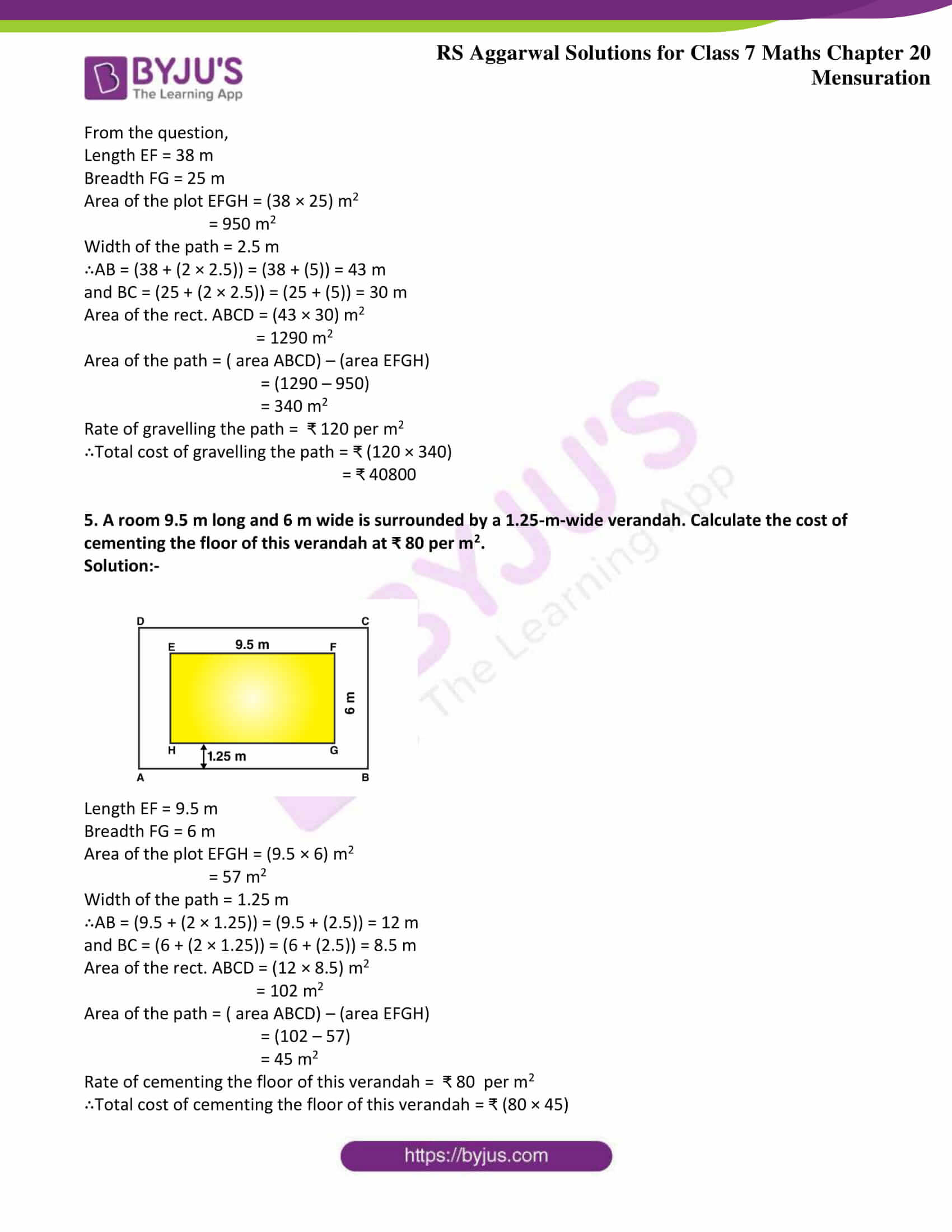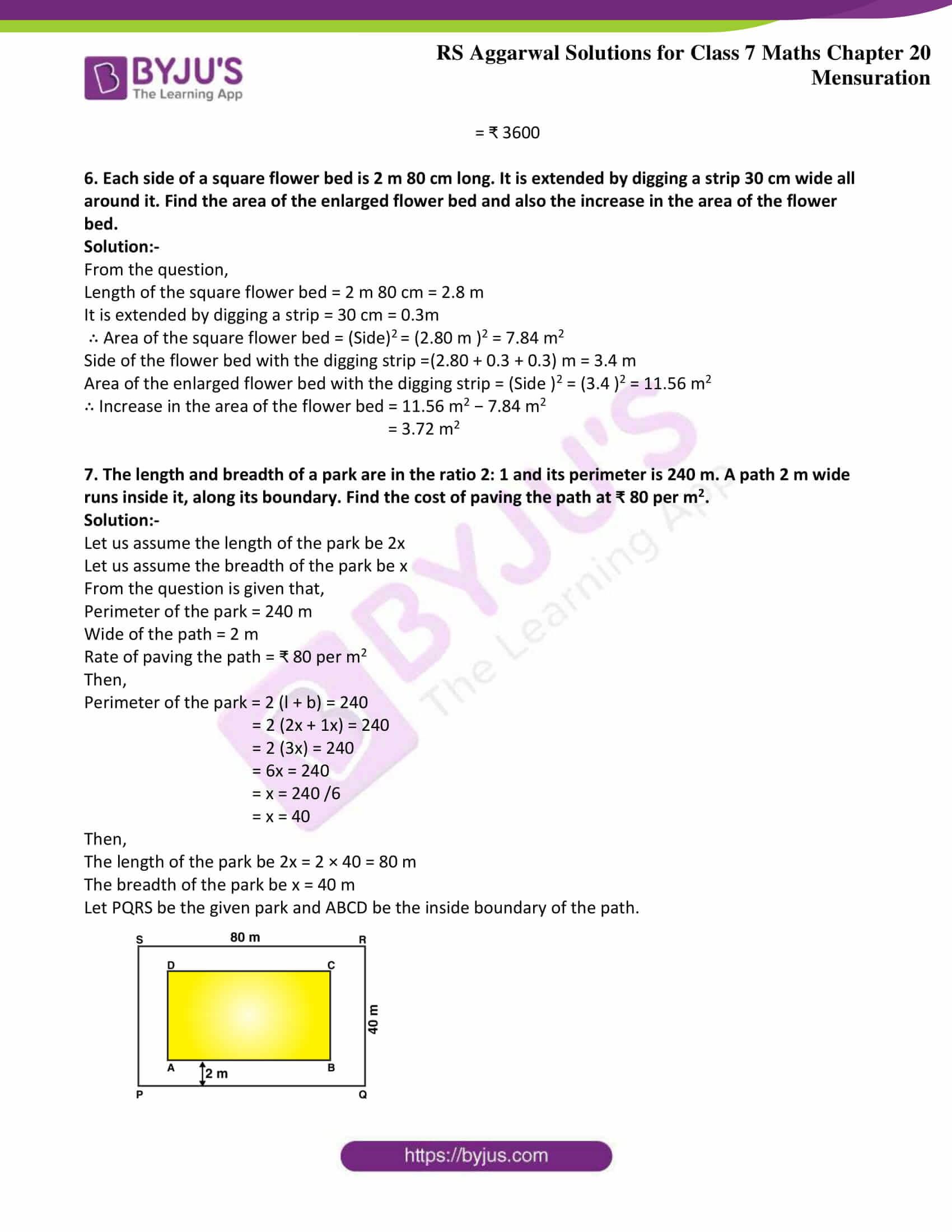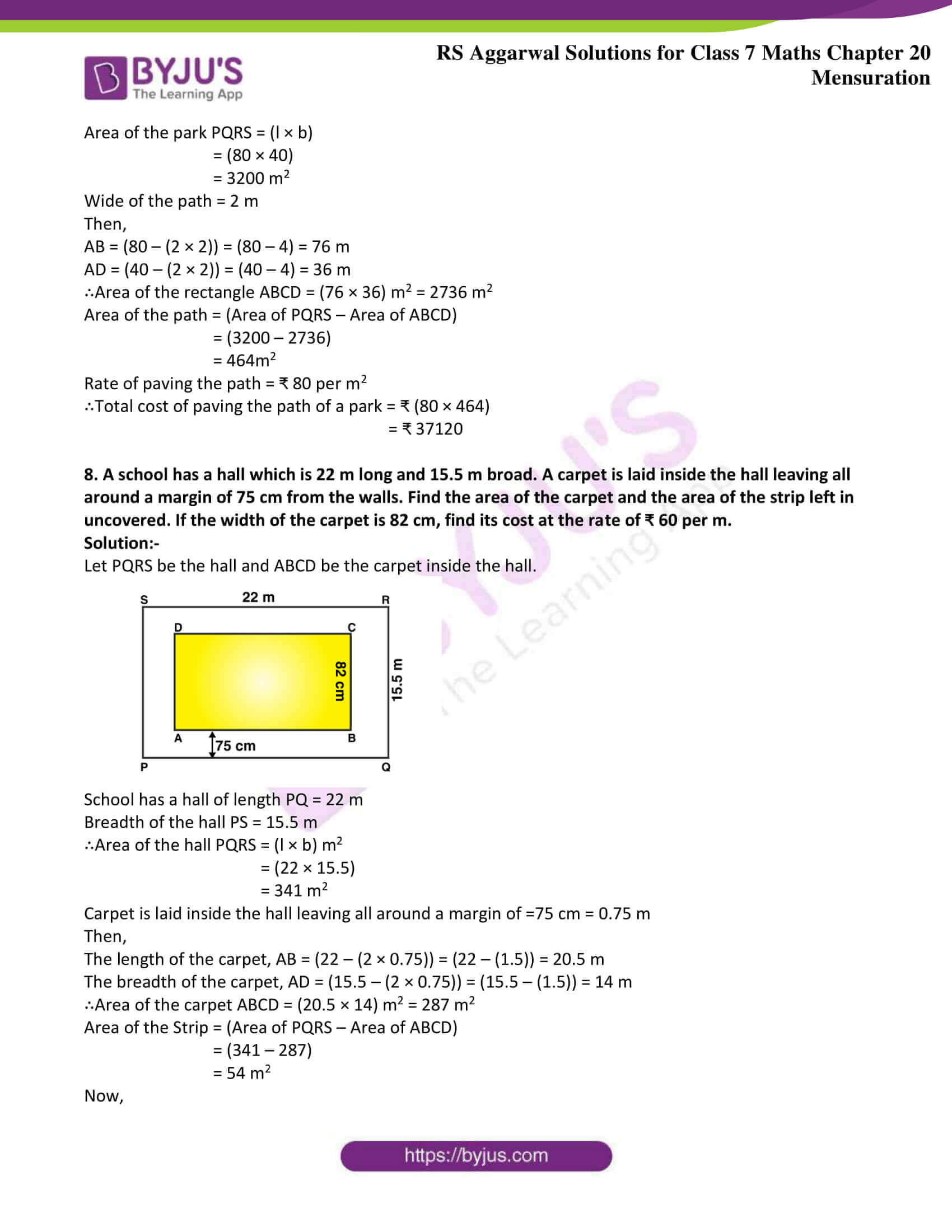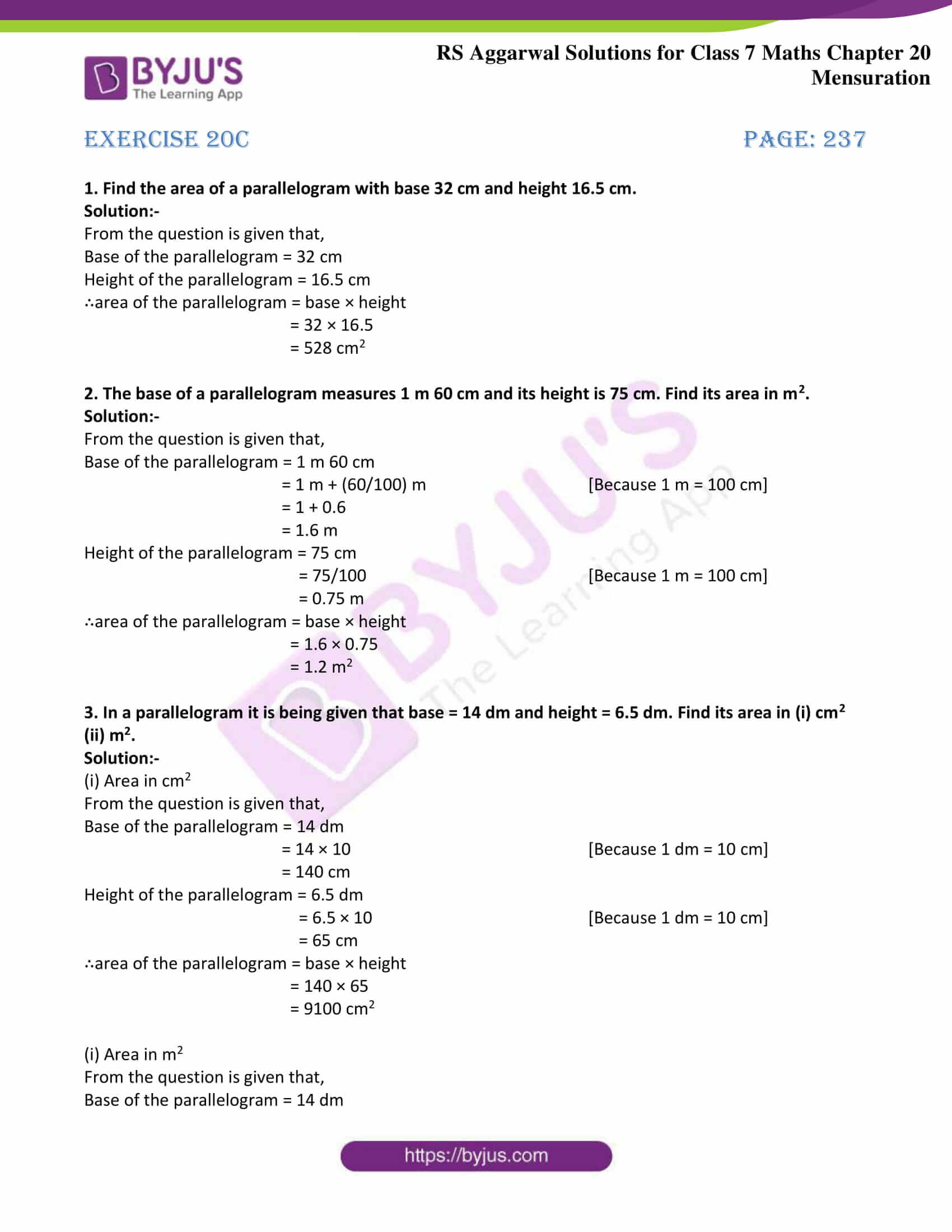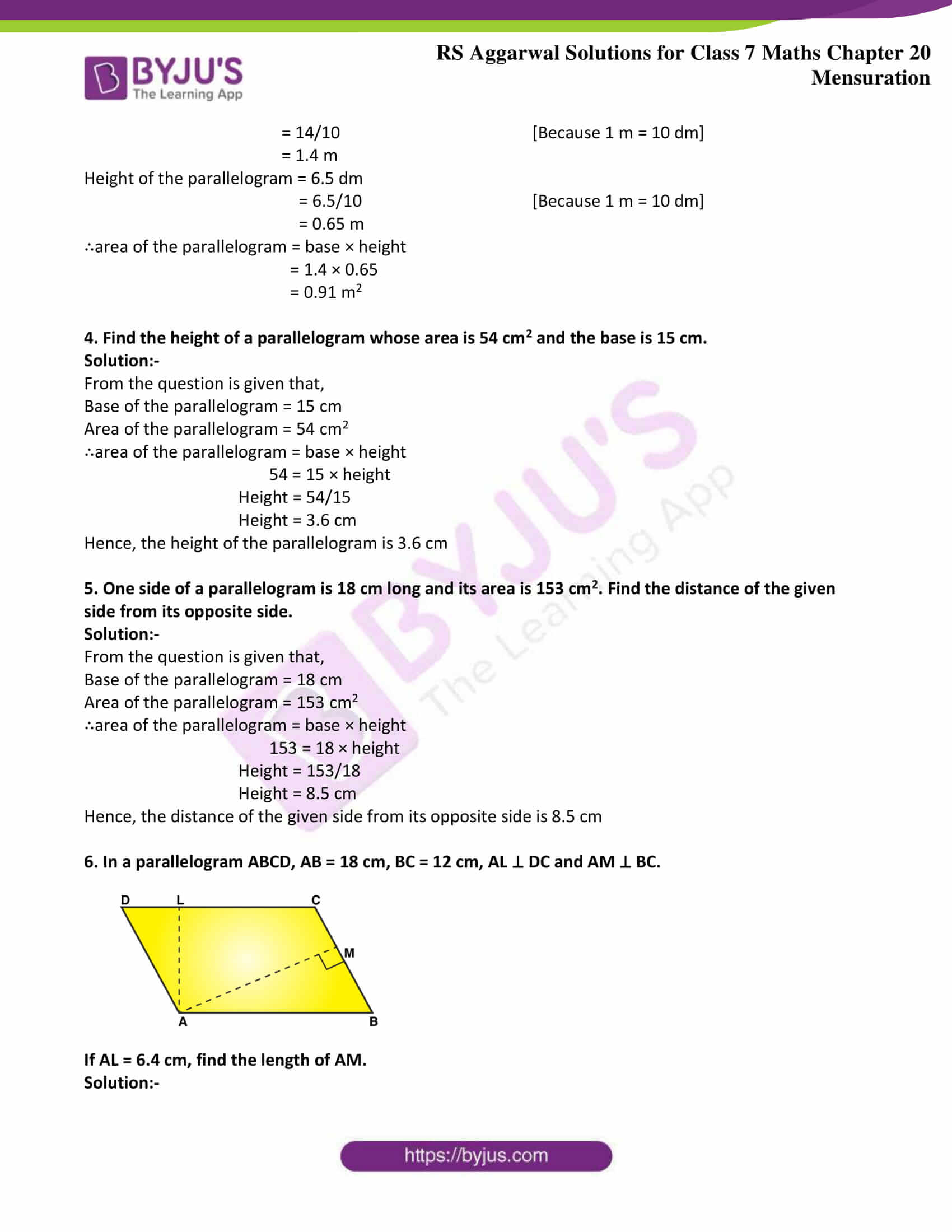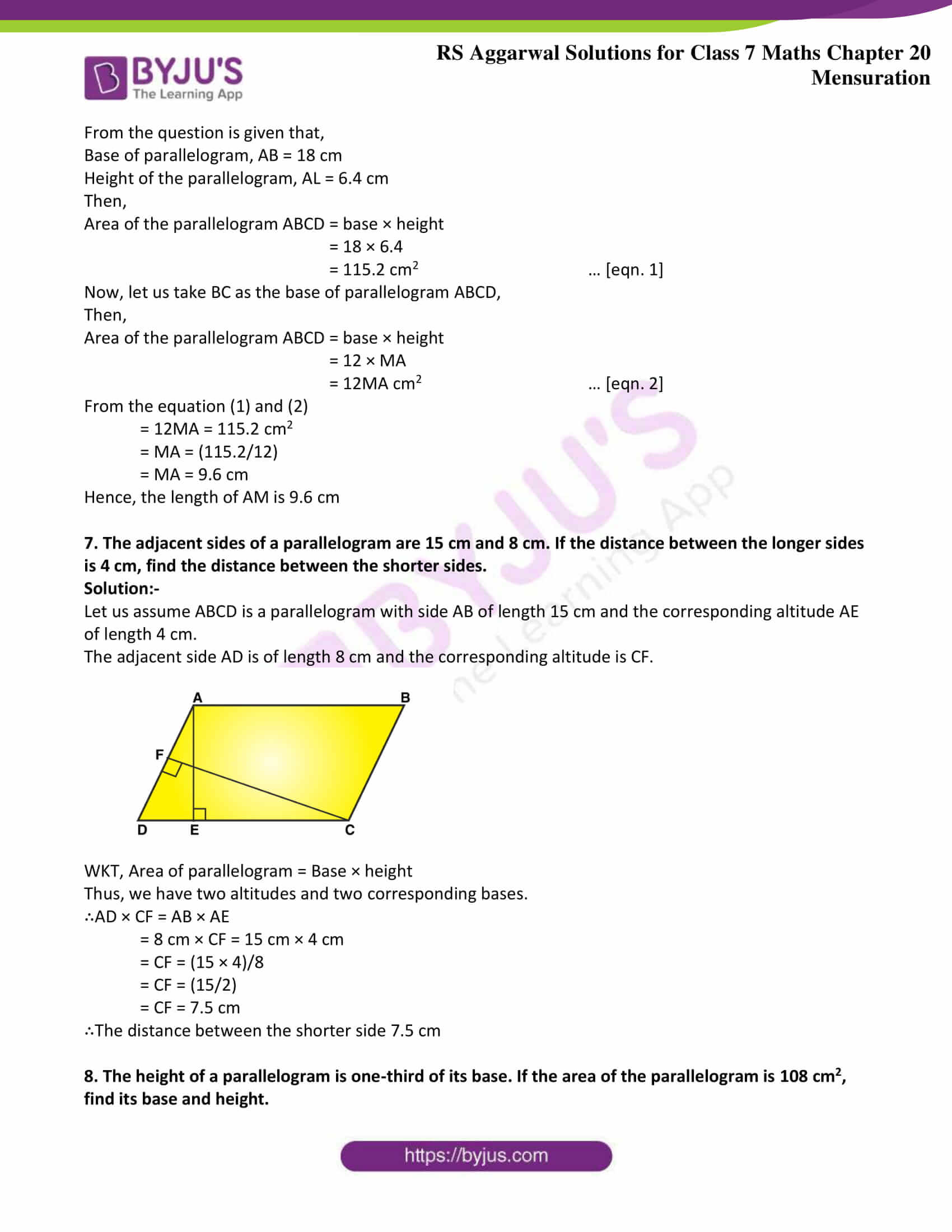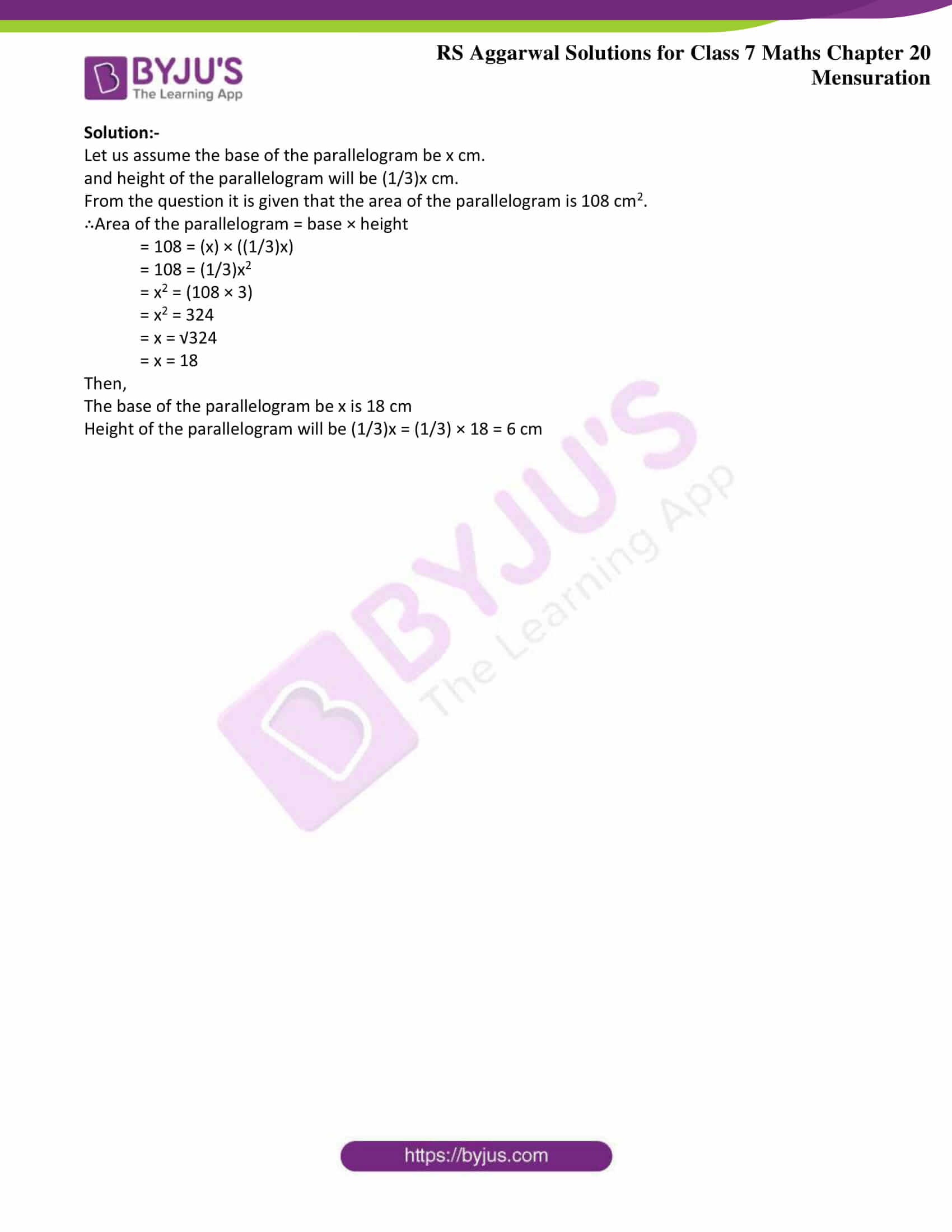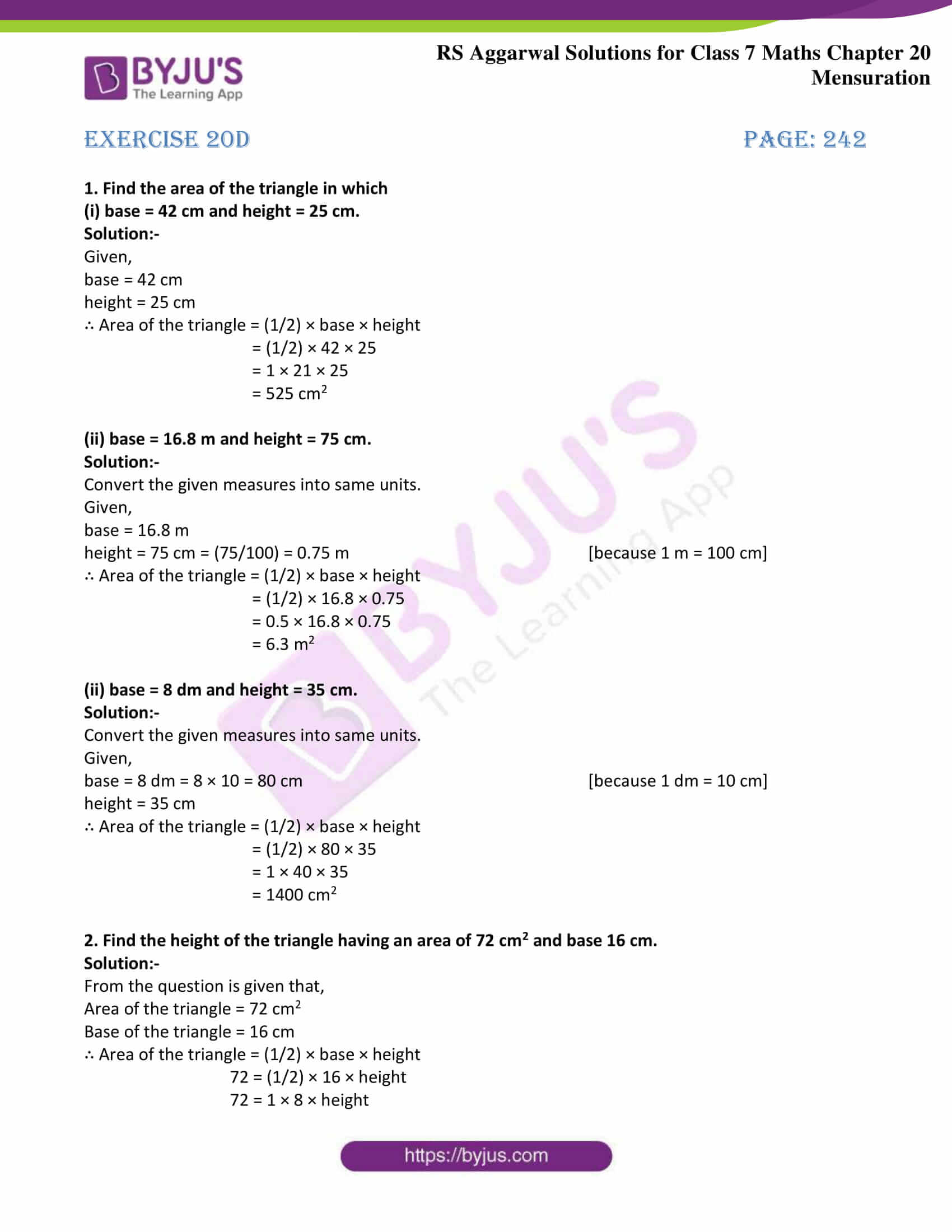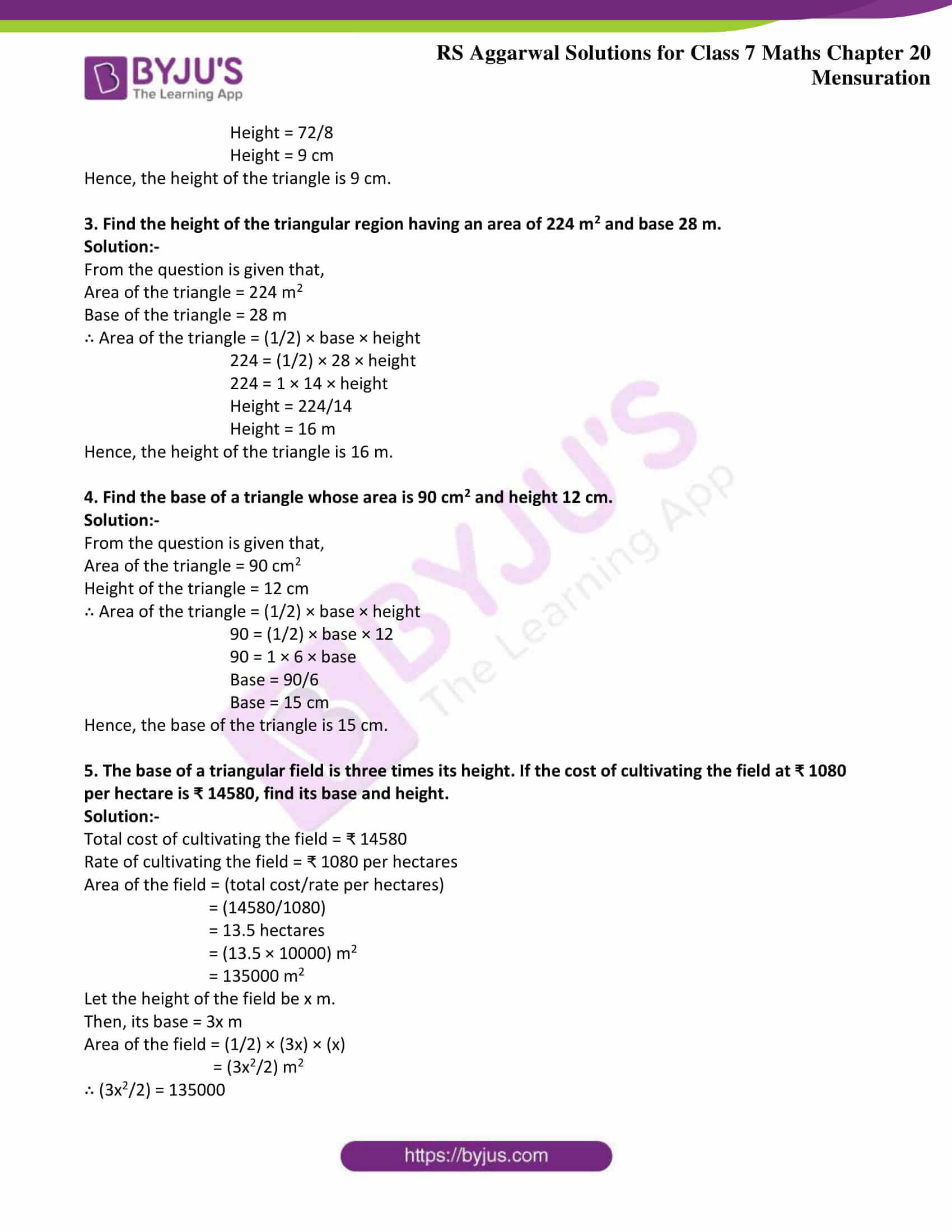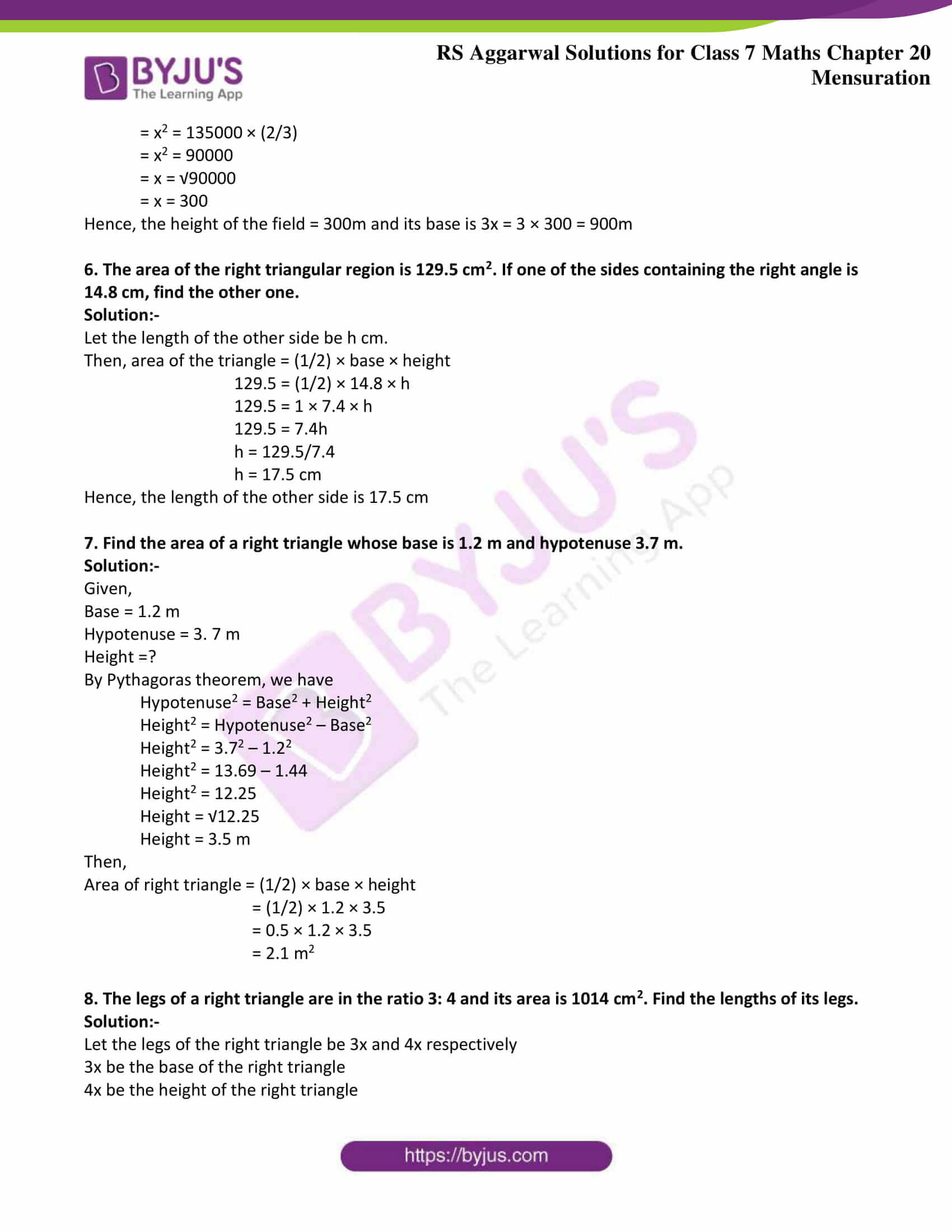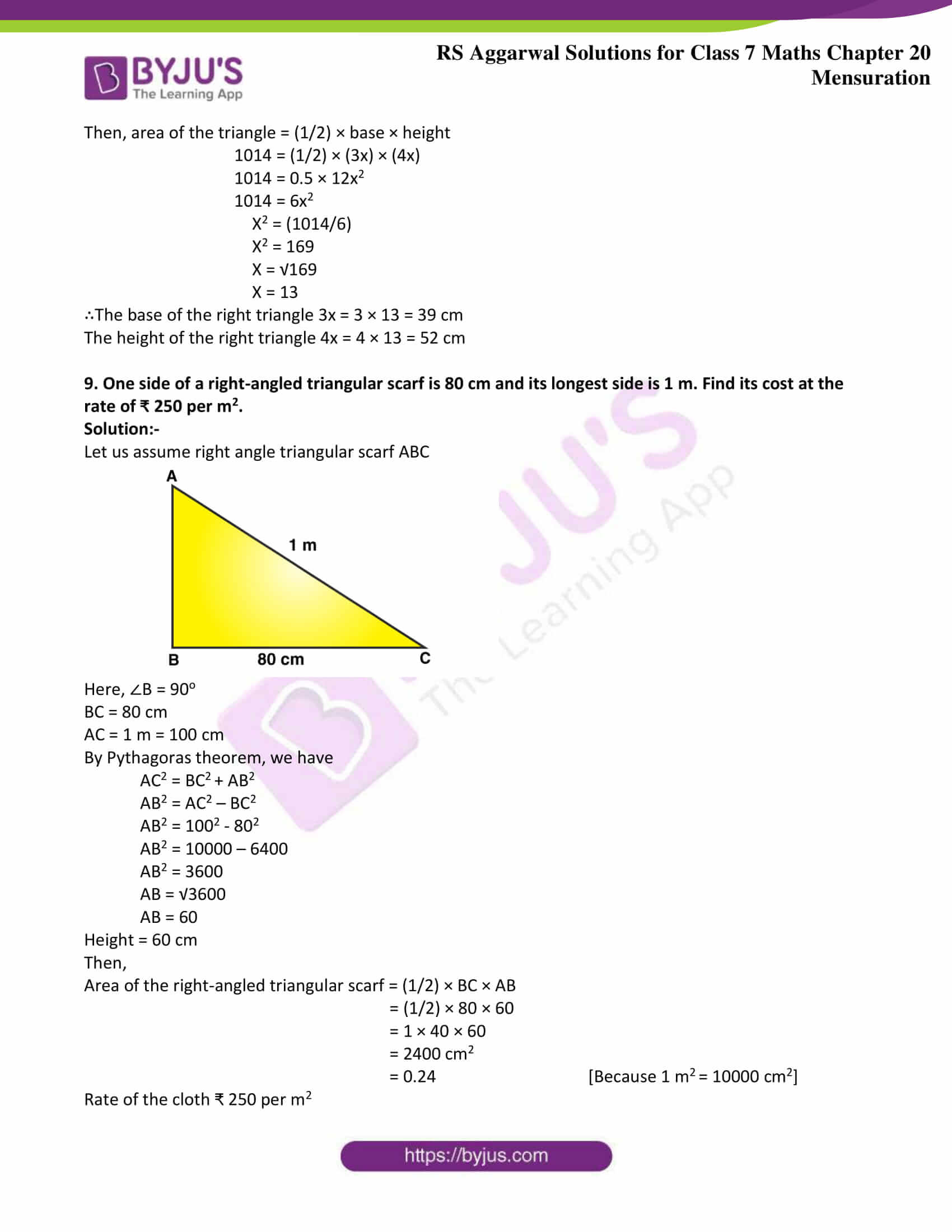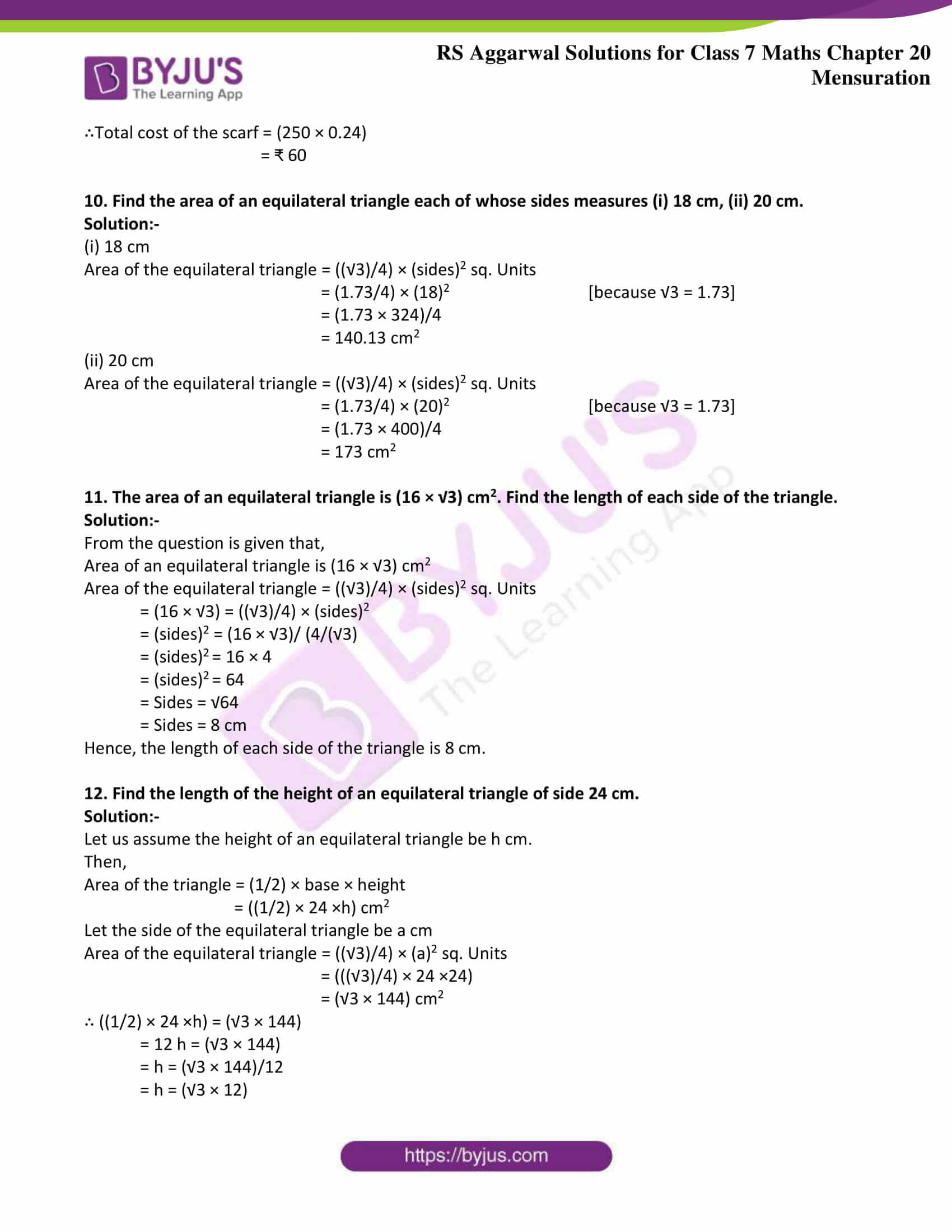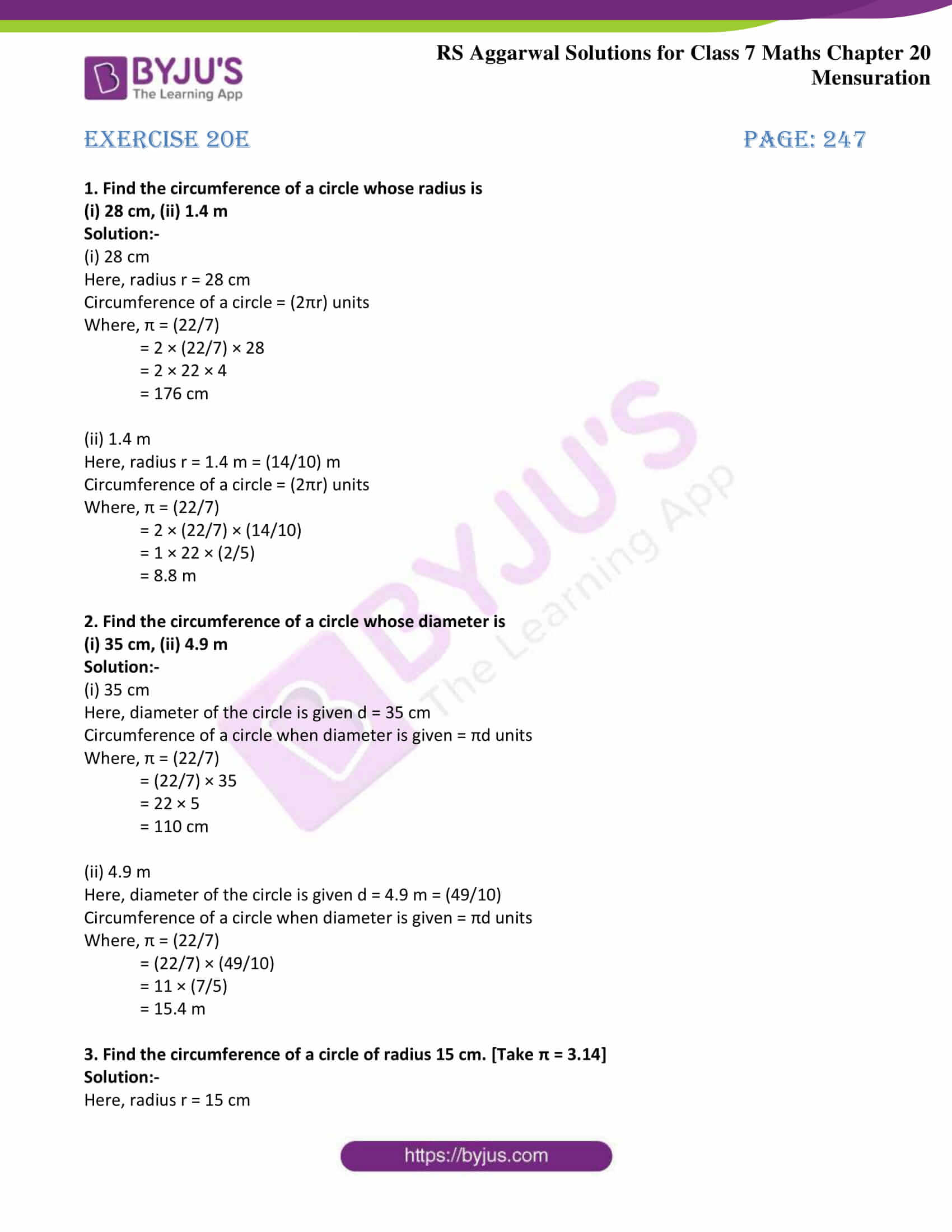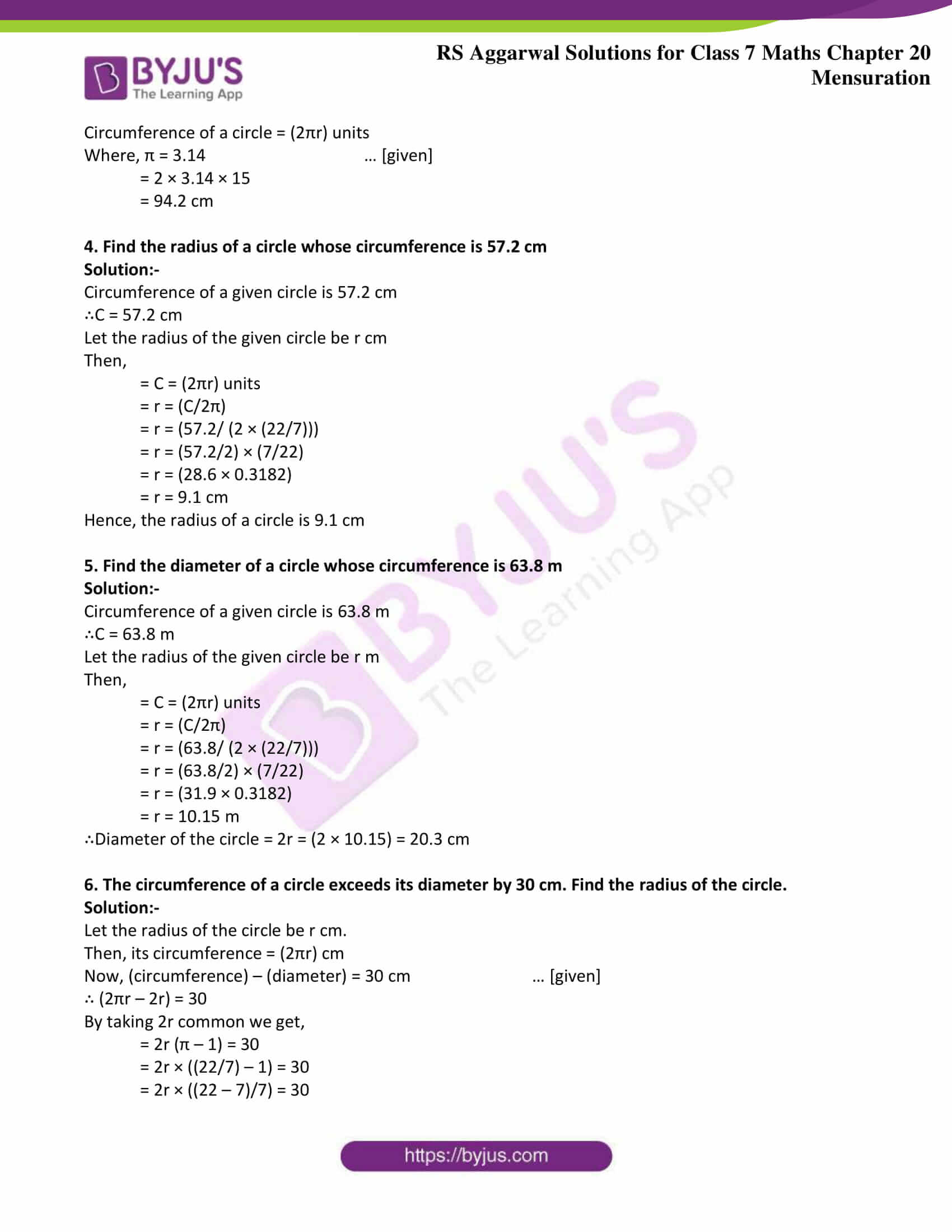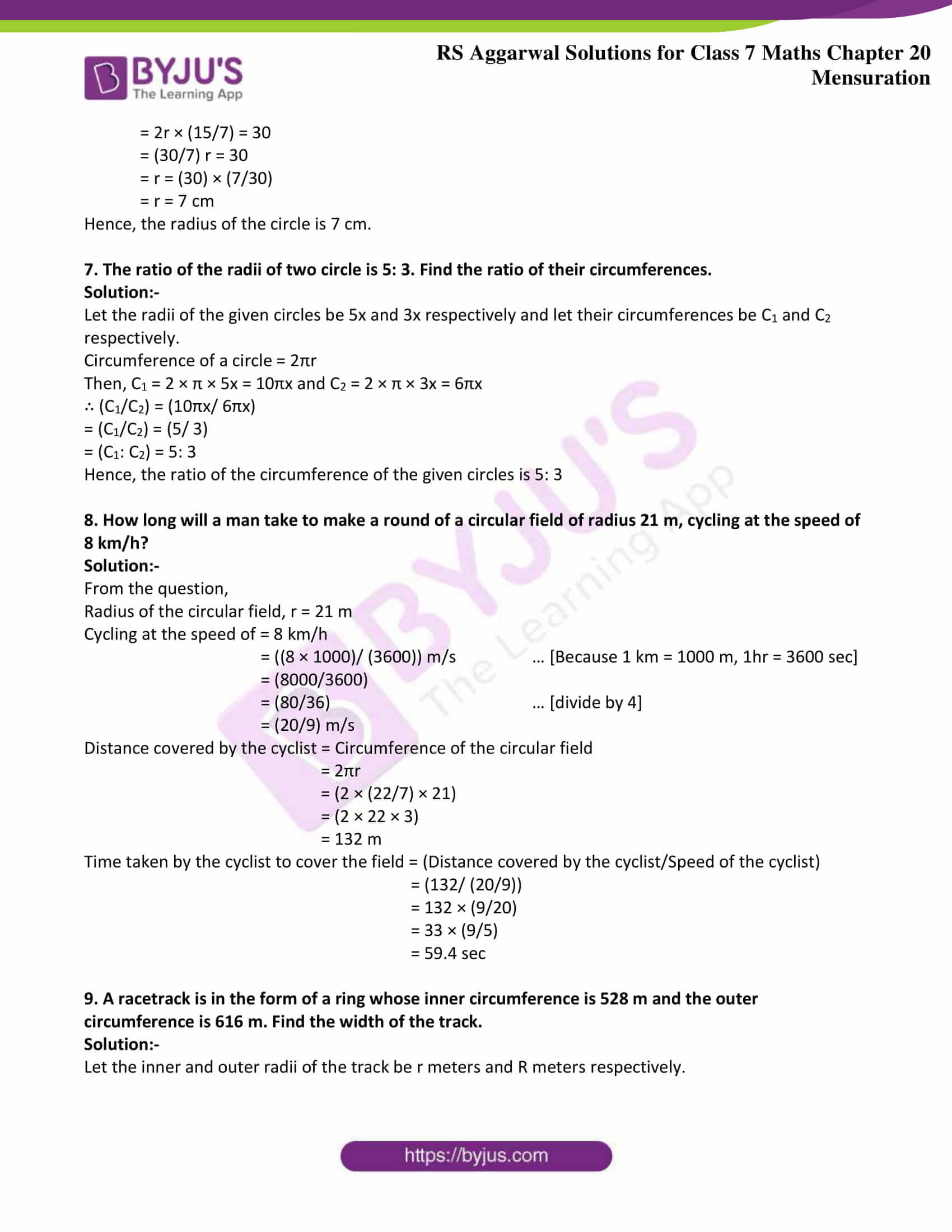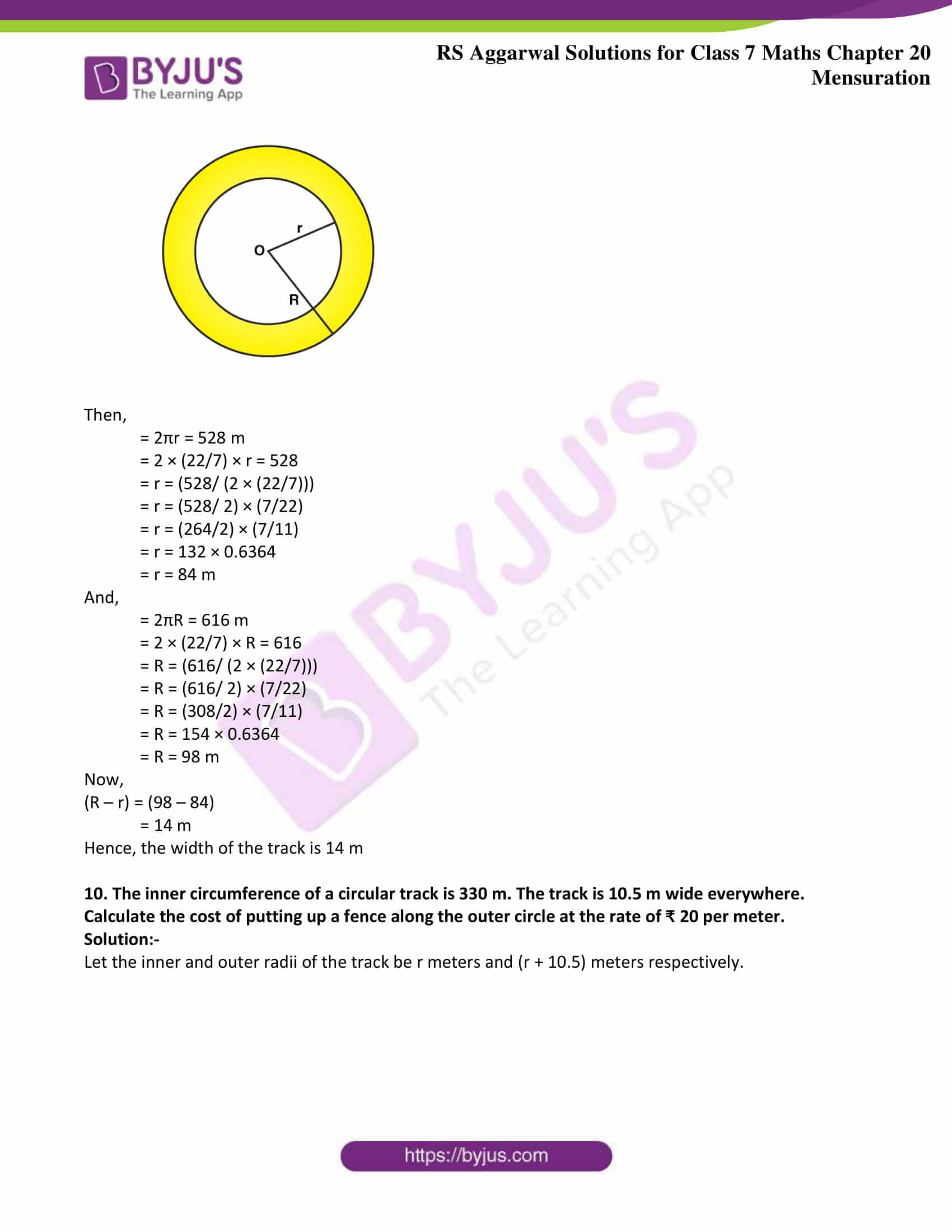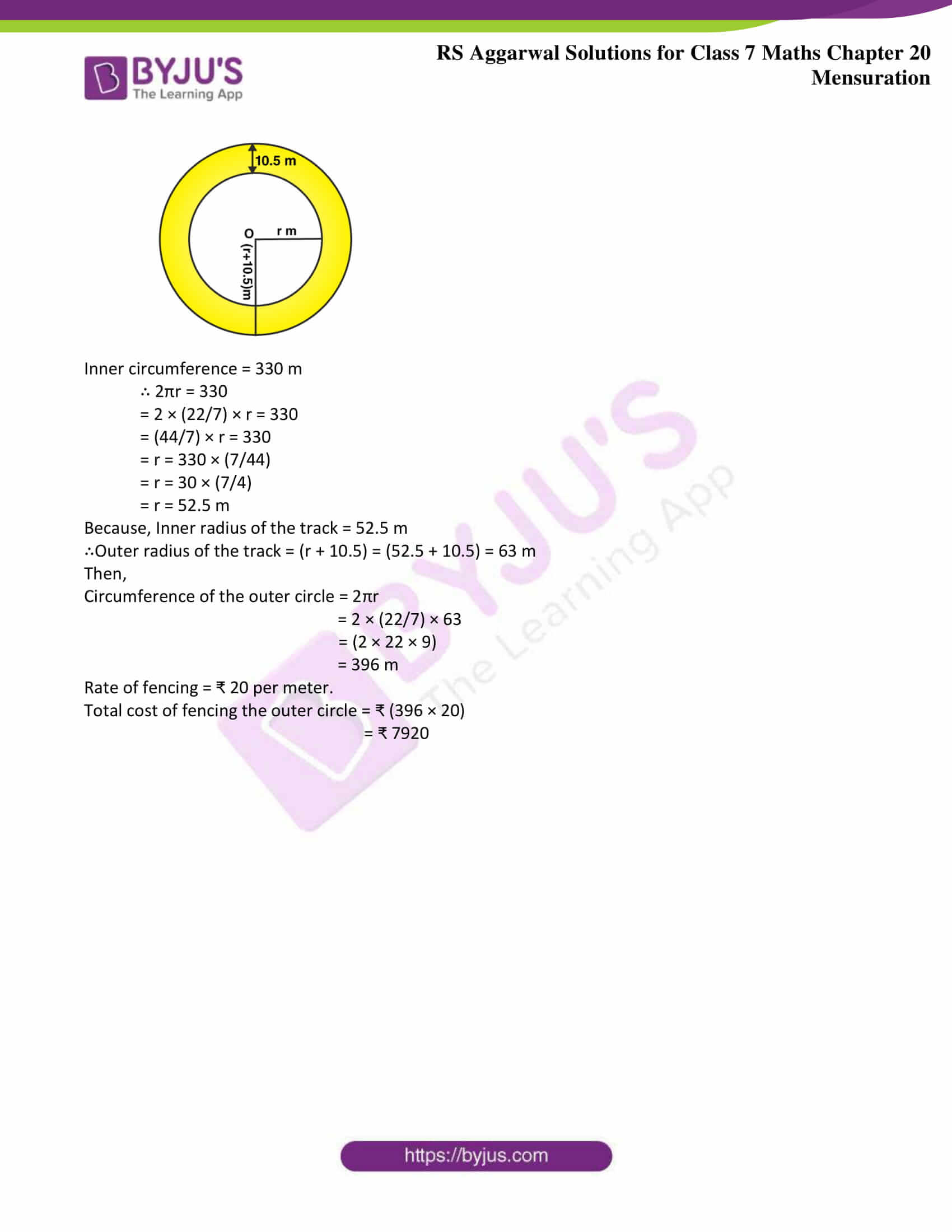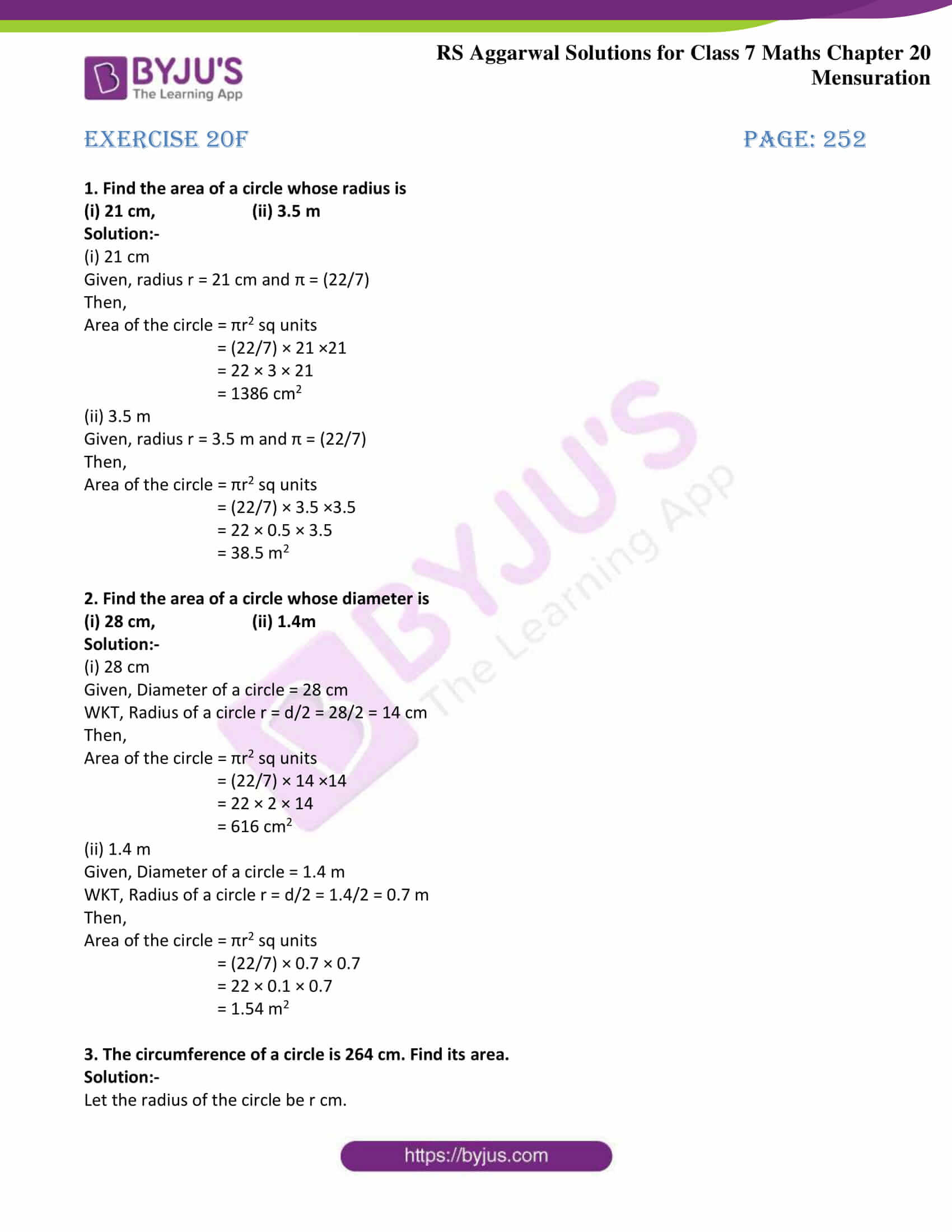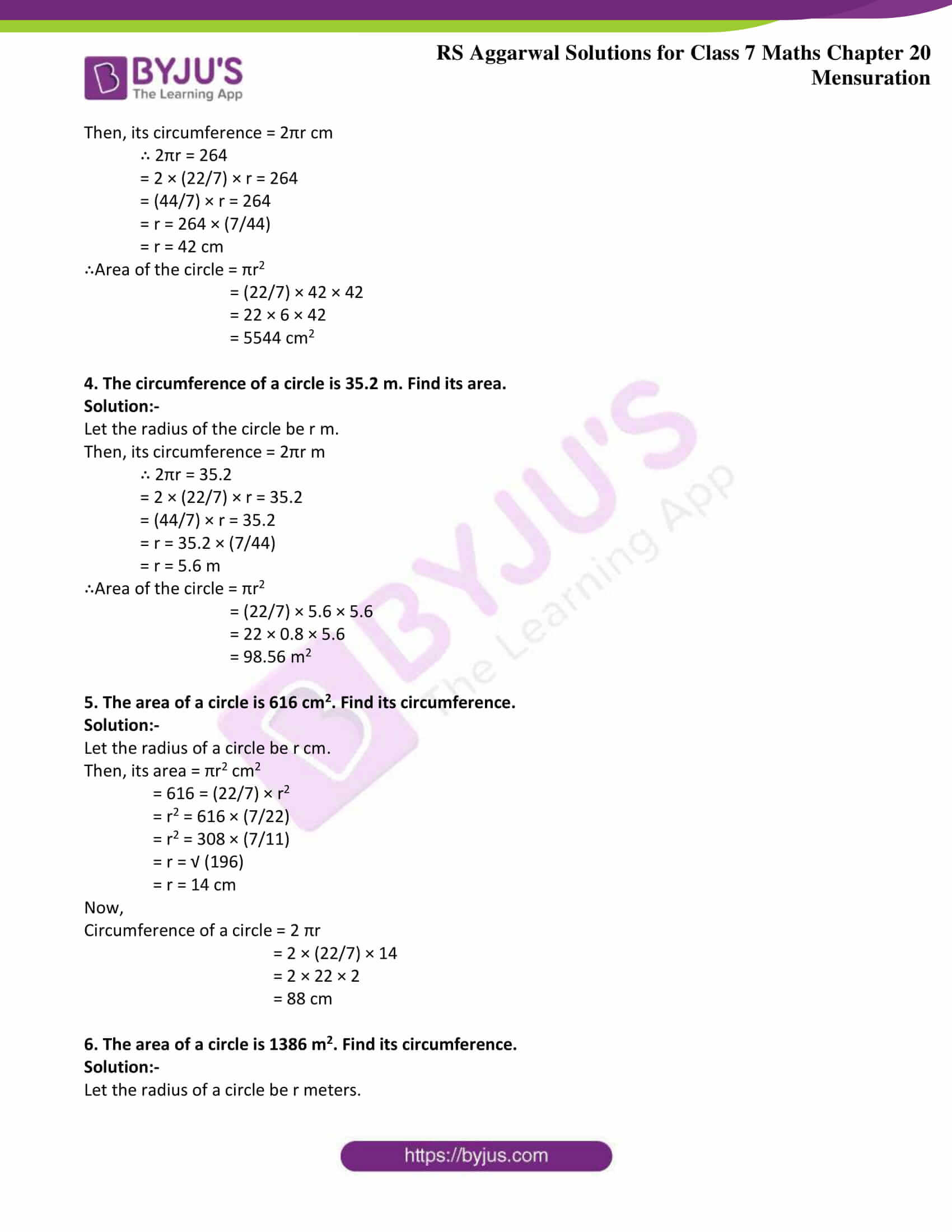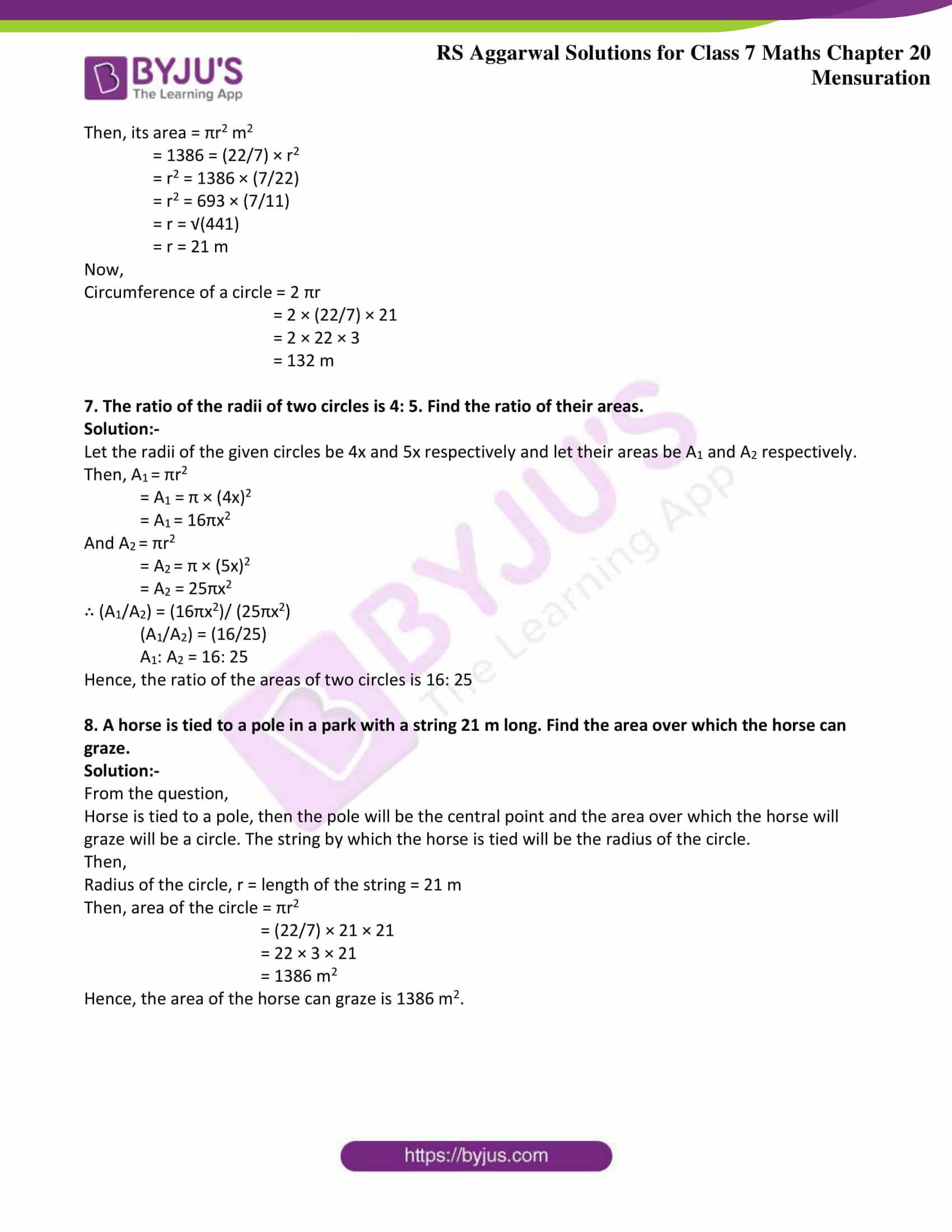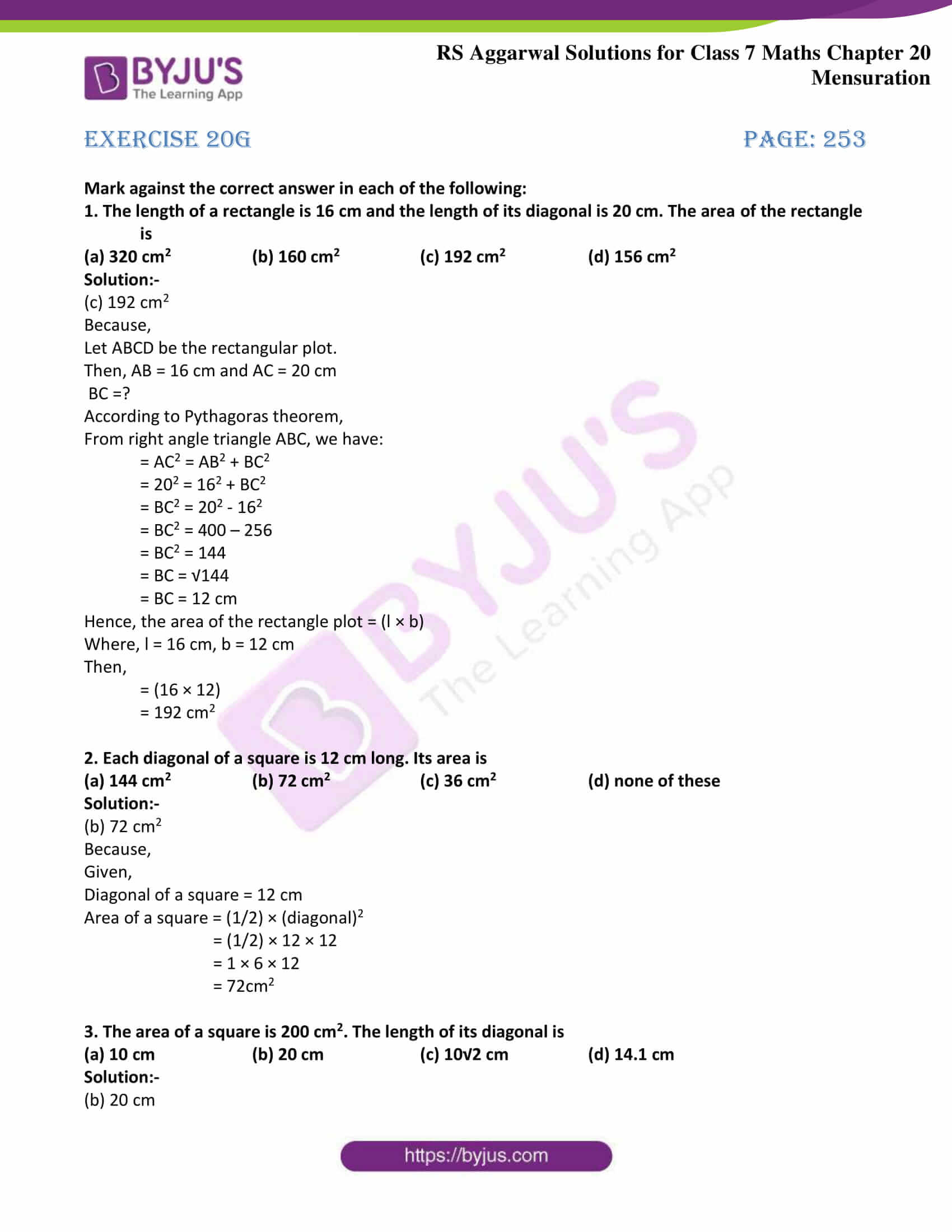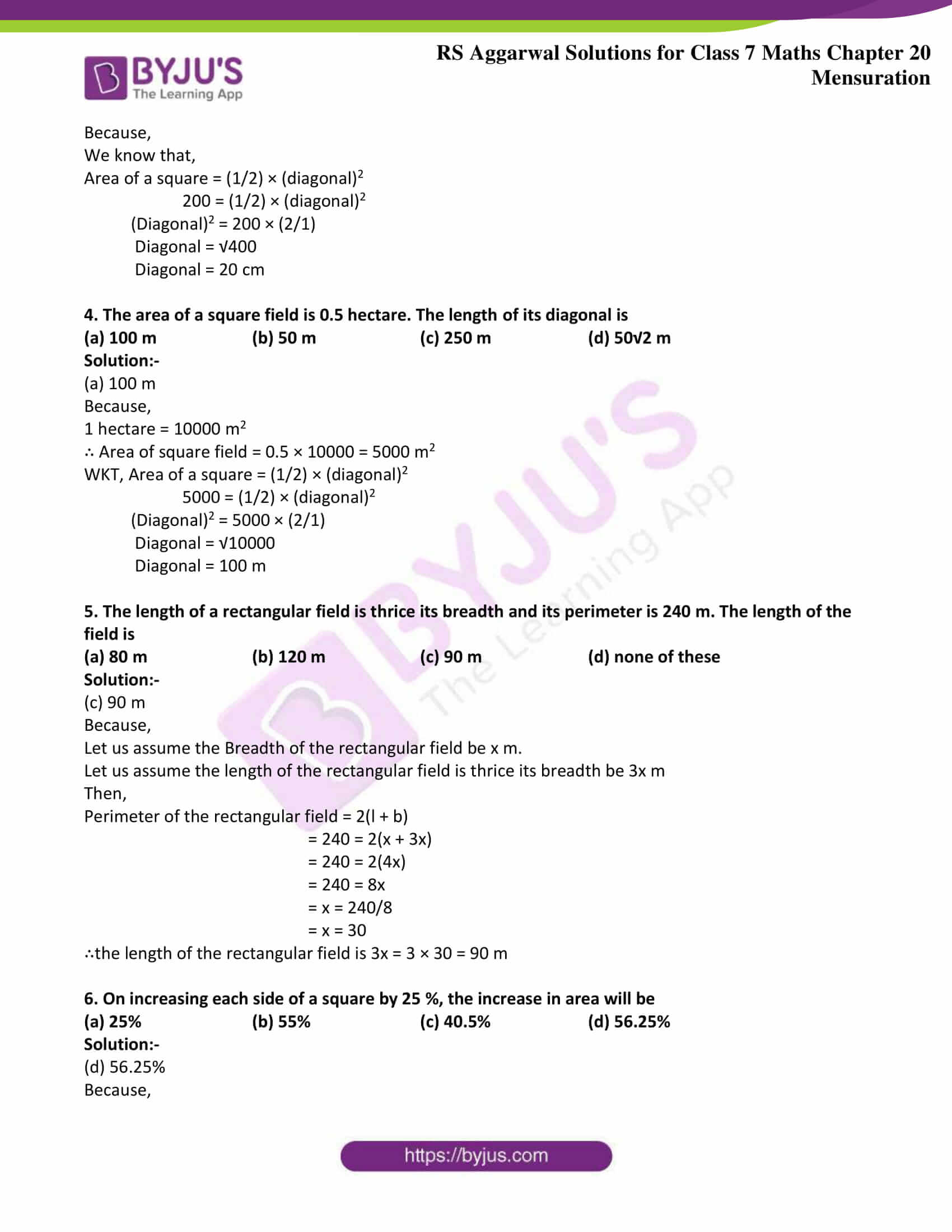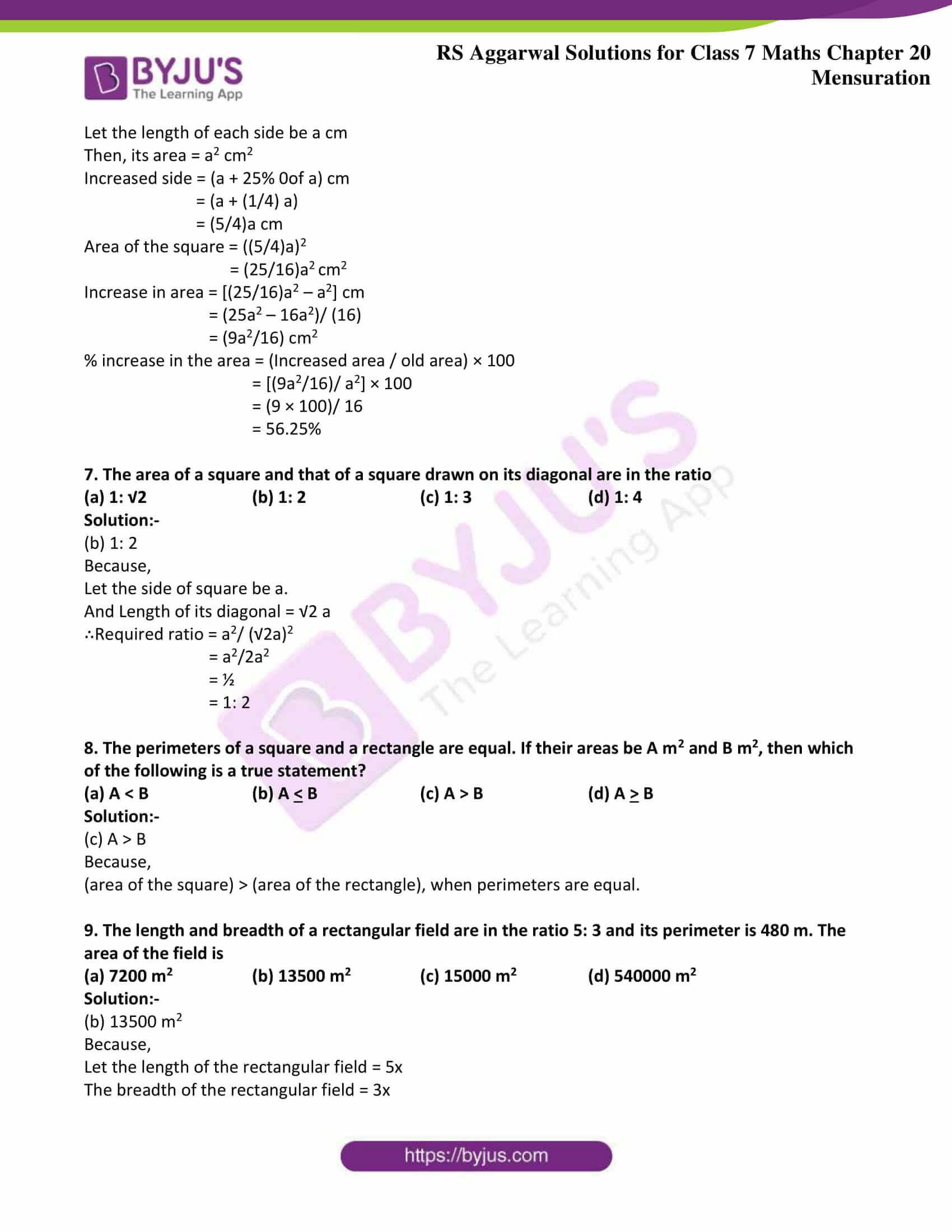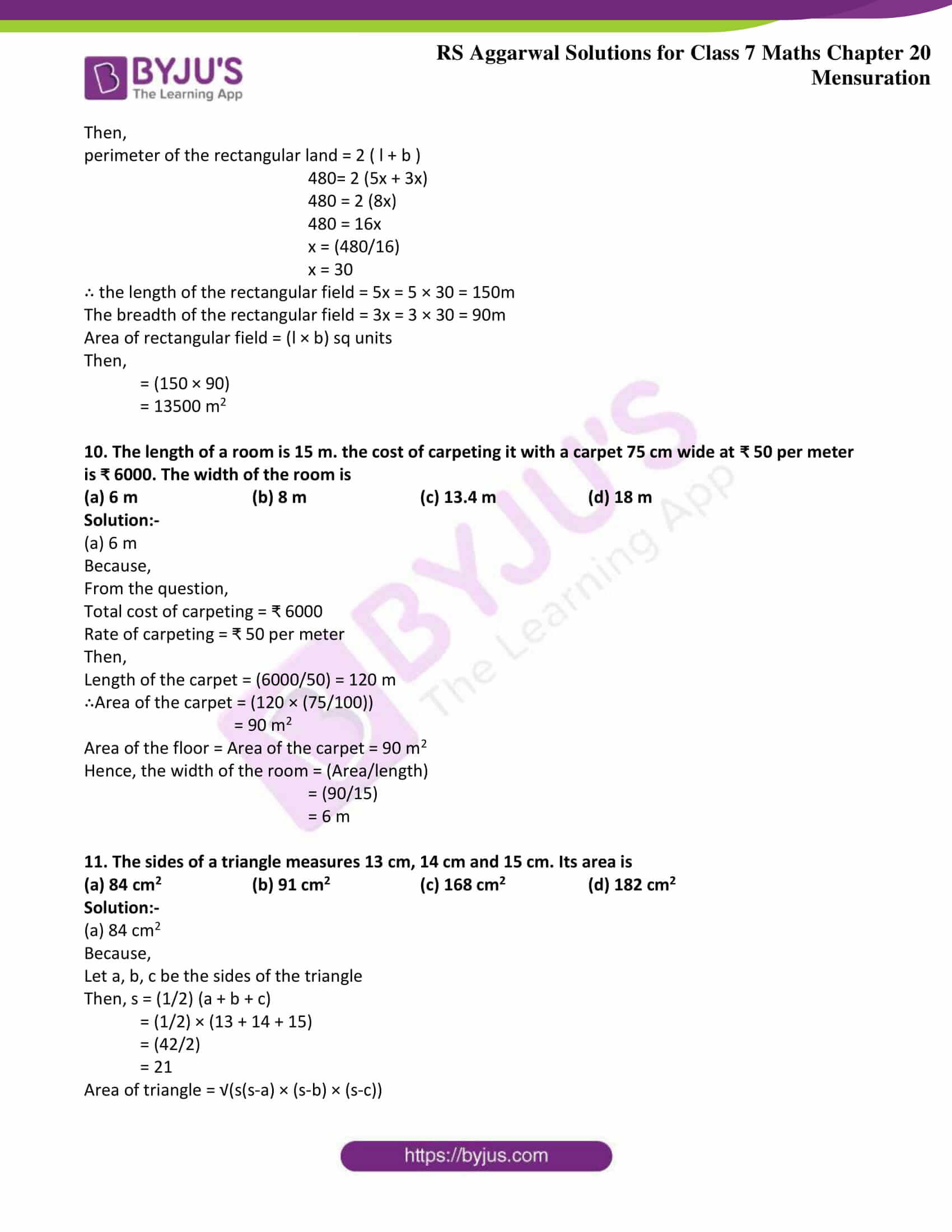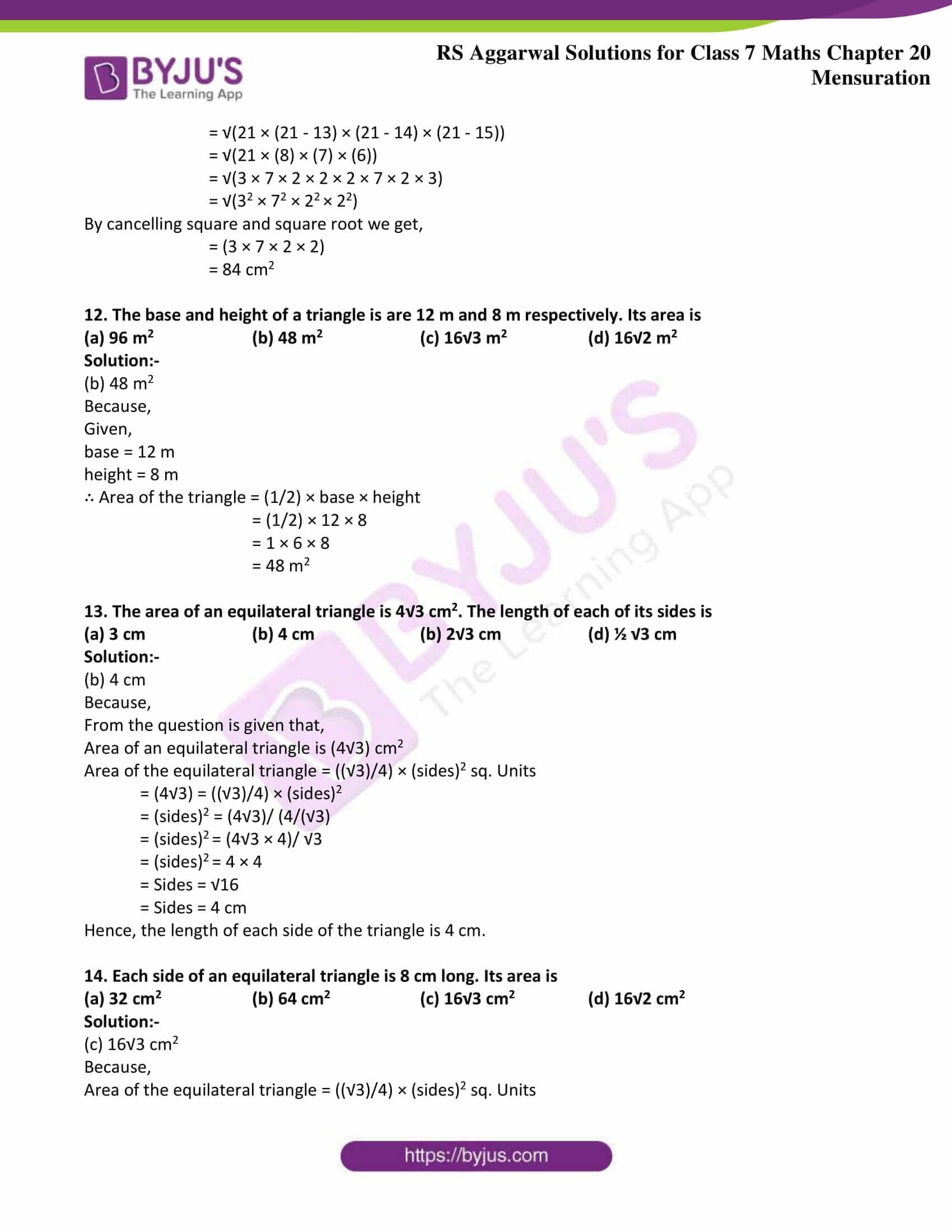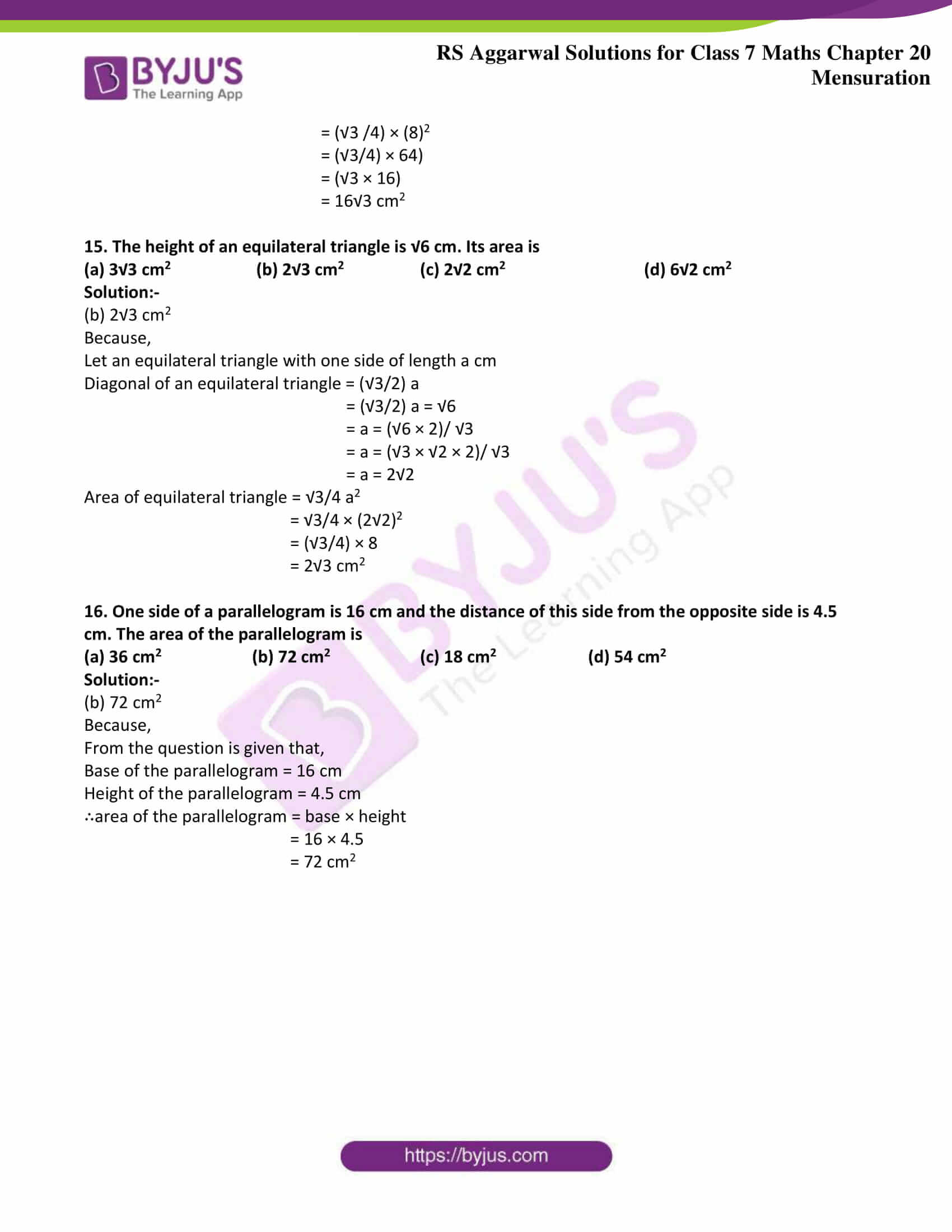Exercise 20A

Exercise 20B

Exercise 20C

Exercise 20D

Exercise 20E

Exercise 20F

Exercise 20G

## Exercise 20A Page: 229

1. Find the area of the rectangle whose dimensions are:

(i) Length = 24.5 m, breadth = 18m

Solution:-

Consider a rectangle with length = l units and breadth = b units. Then, we have:

Area of rectangle = (l × b) sq units

Then,

= (24.5 × 18)

= 441 m2

(ii) Length = 12.5 m, breadth = 8dm

Solution:-

Breadth = 8dm = (8 × 10) = 80cm= 0.8 m [Because 1dm =10 cm and 1m = 100cm]

Consider a rectangle with length = l units and breadth = b units. Then, we have:

Area of rectangle = (l × b) sq units

Then,

= (12.5 × 0.8)

= 10 m2

2. Find the area of a rectangle plot. One side of which is 48 m and its diagonal is 50 m.

Solution:-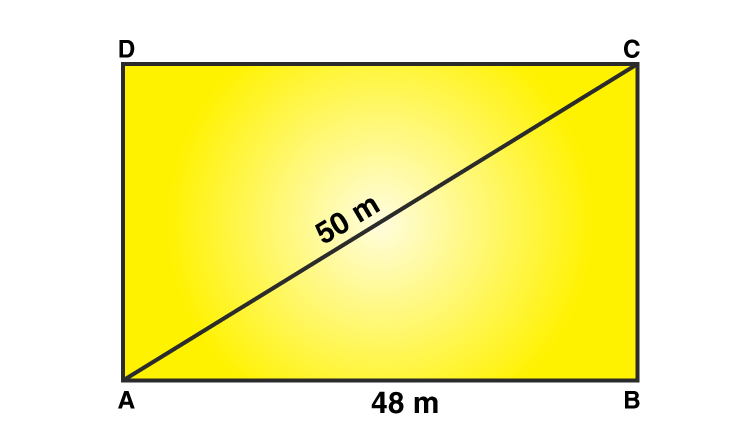Let ABCD be the rectangular plot.

Then, AB = 48 m and AC = 50 m

BC =?

According to Pythagoras theorem,

From right angle triangle ABC, we have:

= AC2 = AB2 + BC2

= 502 = 482 + BC2

= BC2 = 502 – 482

= BC2 = 2500 – 2304

= BC2 = 196

= BC = √196

= BC = 14 m

Hence, the area of the rectangle plot = (l × b)

Where, l = 48 m, b = 14 m

Then,

= (48 × 14)

= 672 m2

3. The sides of a rectangular park are in the ratio 4:3. If its area is 1728 m2, find the cost of fencing it at ₹ 30 per meter.

Solution:-

Let the length of the field be 4x m

∴area of the rectangular park = ((4x) × (3x)) = 12x2 m2

But, area = 1728m2 (from the question)

∴12x2 = 1728

= x2 = 1728/12

= x2 = 144

= x = √144

= x = 12

∴length = (4 × 12) = 48m, breadth = 3 × 12 = 36m

∴perimeter of the field = 2(l + b) units

= 2 (48 + 36)

= 2 (84)

= 168m

∴the cost of fencing = ₹ (168 × 30)

= ₹ 5040

4. The area of a rectangle field is 3584 m2 and its length is 64 m. A boy runs around the field at the rate of 6 km/h. How long will he take to go 5 times around it?

Solution:-

From the question we have,

Area of the rectangular field = 3584 m2

Length of the rectangular field = 64 m

Boy runs around the field at the rate = 6 km/h

= ((6 × 1000) / 60) m/min

= 100 m/min

Breadth of the rectangular field = (area /length)

= (3584/ 64)

= 56 m

∴perimeter of the field = 2(l + b) units

= 2 (64 + 56)

= 2 (120)

= 240m

Total distance covered by the boy = 5 × perimeter of the field

= 5 × 240

= 1200m

∴required time to cover the distance of 1200m = (1200/100) = 12min

Hence, boy took 12min to go 5 times around of rectangular field.

5. A verandah is 40 m long and 15 m broad. It is to be paved with stones, each measuring 6dm by 5dm. Find the number of stones required.

Solution:-

Convert all the given measures into same units.

From the question,

Verandah has length of = 40 m = (40 × 10) dm = 400 dm [Because 1m = 10dm]

Verandah has length of = 15 m = (15 × 10) dm = 150 dm

∴Area of the verandah = (l × b)

= (400 × 150) dm2

= 60000 dm2

Now,

Length of a stone = 6dm (given)

Breadth of a stone = 5dm

∴Area of a stone = (l × b)

= (6 ×5)

= 30 dm2

∴Total number of stones required to pave the verandah = (Area of verandah/ Area of each stone)

= (60000/ 30)

= 2000 stones

6. Find the cost of carpeting a room 13 m by 9 m with a carpet of width 75 cm at the rate of ₹ 105 per meter.

Solution:-

From the question,

Length of room = 13 m

Width of the carpet = 75 cm = (75/100) m = 0.75m

The rate of carpeting the room = ₹ 105 per meter.

∴Area of the room = (l × b)

= (13 × 9)

= 117m2

Length of the carpet = (Area of the carpet / Width of the carpet)

By the question we understood that, Area of the room = Area of the carpet

Then,

= (Area of the carpet / Width of the carpet)

= (117/0.75)

= 156 m

∴Total cost of carpeting = ₹ (156 × 105)

= ₹ 16380

7. The cost of carpeting a room 15 m long with a carpet of width 75 cm at ₹ 80 per meter is ₹ 19200. Find the width of the room.

Solution:-

From the question,

Length of the room = 15 m

Width of the carpet = 75 cm = (75/100)m = 0.75 m

Cost of carpet = ₹ 80

Cost of carpeting the room = ₹ 19200

Let the length of the carpet be x m

∴Cost of x m carpet = ₹ (80 × x) = ₹ 80x

Then,

Cost of carpeting the room = ₹ 19200

= 80x = 19200

= x = 19200/80

= x = 240

∴The length of the carpet required for carpeting the room is 240m

Then,

Area of the carpet required for carpeting the room = Length of the carpet × Width of the carpet

= (240 × 0.75) m2

= 180m2

Now,

We have to find width of the room be b m.

Carpeting area = 15 m × b m

= 15b m2

∴ 15b m2 = 180 m2

= b = (180/15) m

= b = 12 m

Hence, the width of the room is 12 m

8. The length and breadth of a rectangular piece of land are in the ratio of 5: 3. If the total cost of fencing it at ₹ 24 per mater is ₹ 9600, find its length and breadth.

Solution:-

From the question,

The cost of fence = ₹ 24 per mater

The total cost of fencing a rectangular piece of land = ₹ 9600

Let the length of the rectangular field = 5x

The breadth of the rectangular field = 3x

Then,

perimeter of the rectangular land = 2 ( l + b )

= 2 (5x + 3x)

= 2 (8x)

= 16x

Perimeter of the given field = (total cost of fencing / Rate of fencing)

16x = (9600/24)m

16x = 400m

x = 400/16

x = 25

∴ the length of the rectangular field = 5x = 5 × 25 = 125m

The breadth of the rectangular field = 3x = 3 × 25 = 75m

9. Find the length of the largest pole that can be placed in a hall 10 m long, 10 m wide and 5 m high.

Solution:-

From the question we conclude that the hall is in rectangular shape.

In that hall the largst pole means, the diagonal

∴let The length of the diagonal of the room = √(l2 + b2 + h2)

= √(102 +102 + 52)

= √(100+ 100 + 25)

= √(225)

= 15m

Hence the length of the diagonal of the room is 15m

10. Find the area of a square each of whose sides measures 8.5m.

Solution:-

From the question,

Each sides of square measures = 8.5 m

Then,

Area of the square = a2 units

= (8.5)2

= 72.25 m2

## Exercise 20B Page: 232

1. A rectangular grassy plot is 75 m long and 60 m broad. It has a path of width 2 m all around it on the inside. Find the area of the path and the cost of constructing it at ₹ 125 per m2.

Solution:-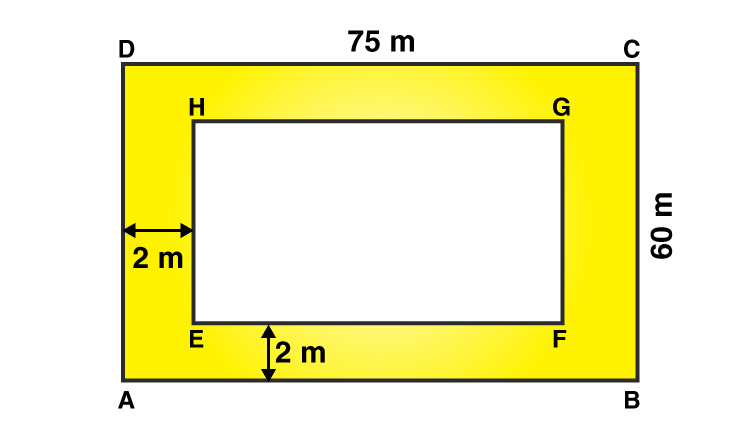Let ABCD be the given grassy plot and let EFGH be the inside boundary of the path,

Then,

Length AB = 75 m

Area of the plot ABCD = (75 × 60) m2

= 4500 m2

Width of the path = 2 m

∴EF = (75 – (2 × 2)) = (75 – (4)) =71 m

and FG = (60 – (2 × 2)) = (60 – (4)) = 56 m

Area of the rect. EFGH = (71 × 56) m2 = 3976 m2

Area of the gravel path = ( area ABCD) – (area EFGH)

= (4500 – 3976)

= 524 m2

Hence, cost of constructing the path = ₹ (524 × 125)

= ₹ 65500

2. A rectangle plot of land measures 95 m by 72 m. Inside the plot, a path of uniform width of 3.5 m is to be constructed all around. He rest of of the plot is to be laid with grass. Find the total expenses involved in constructing the path at ₹ 80per m2 and laying the grass at ₹ 40 per m2.

Solution:-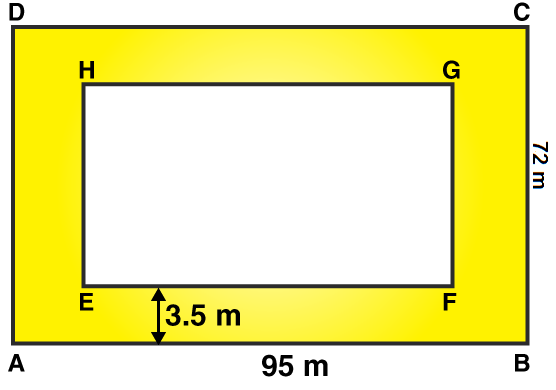Let ABCD be the given grassy plot and let EFGH be the inside boundary of the path,

Then,

Length AB = 95 m

Area of the plot ABCD = (95 × 72) m2

= 6840 m2

Width of the path = 3.5 m

∴EF = (95 – (2 × 3.5)) = (95 – (7)) =88 m

and FG = (72 – (2 × 3.5)) = (72 – (7)) = 65 m

Area of the rect. EFGH = (88 × 65) m2

= 5720 m2

Area of the gravel path = ( area ABCD) – (area EFGH)

= (6840 – 5720)

= 1120 m2

Rate of constructing the path = ₹ 80 per m2

∴Total cost of constructing the path = ₹ (1120 × 80)

= ₹ 89600

Rate of laying the grass on the plot EFGH = ₹ 40 per m2

∴Total cost of laying the grass on the plot = ₹ (5720 × 40)

= ₹ 228800

∴ the total expenses involved in constructing the path = ₹ (89600 + 228800)

= ₹ 318400

3. A saree is 5 m long and 1.3 m wide. A border of width 25 cm is printed along its sides. Find the cost of printing the border at ₹ 1 per 10 cm2.

Solution:-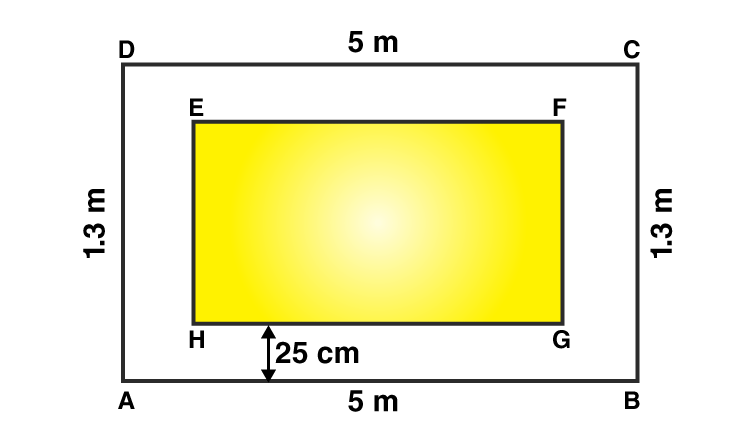Let ABCD be the saree and let EFGH be the part without border,

Then,

Length AB = 5 m

Area of the plot ABCD = (5 × 1.3) m2

= 6.5 m2

Width of the path = 25 cm = (25/100) = 0.25 m

∴EF = (5 – (2 × 0.25)) = (5 – (0.5)) =4.5 m

and FG = (1.3 – (2 × 0.25)) = (1.3 – (0.5)) = 0.8 m

Area of the rect. EFGH = (4.5 × 0.8) m2

= 3.6 m2

Area of the border = ( area ABCD) – (area EFGH)

= (6.5 – 3.6)

= 2.9 m2

= 2.9 × 1000

= 29000 cm2

Rate of printing the Border = ₹ 1 per 10 cm2

∴Total cost of printing the border = ₹ (1 × 29000)/10

= ₹ 2900

4. A rectangular grassy lawn measuring 38 m by 25 m has been surrounded externally by a 2.5-m-wide path. Calculate the cost of gravelling the path at the rate of ₹ 120 per m2.

Solution:-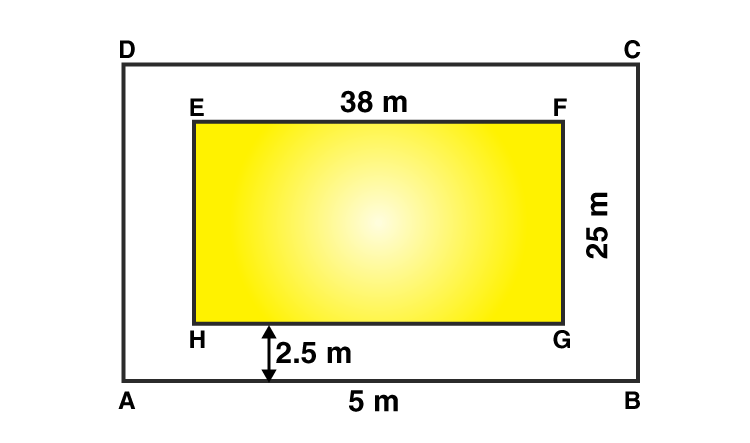From the question,

Length EF = 38 m

Area of the plot EFGH = (38 × 25) m2

= 950 m2

Width of the path = 2.5 m

∴AB = (38 + (2 × 2.5)) = (38 + (5)) = 43 m

and BC = (25 + (2 × 2.5)) = (25 + (5)) = 30 m

Area of the rect. ABCD = (43 × 30) m2

= 1290 m2

Area of the path = ( area ABCD) – (area EFGH)

= (1290 – 950)

= 340 m2

Rate of gravelling the path = ₹ 120 per m2

∴Total cost of gravelling the path = ₹ (120 × 340)

= ₹ 40800

5. A room 9.5 m long and 6 m wide is surrounded by a 1.25-m-wide verandah. Calculate the cost of cementing the floor of this verandah at ₹ 80 per m2.

Solution:-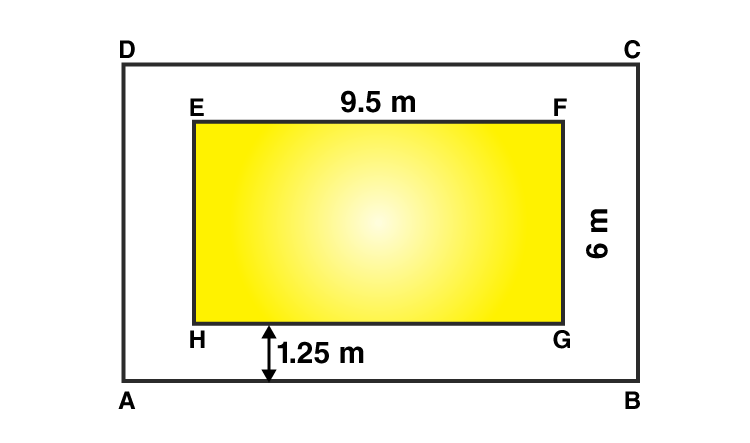Length EF = 9.5 m

Area of the plot EFGH = (9.5 × 6) m2

= 57 m2

Width of the path = 1.25 m

∴AB = (9.5 + (2 × 1.25)) = (9.5 + (2.5)) = 12 m

and BC = (6 + (2 × 1.25)) = (6 + (2.5)) = 8.5 m

Area of the rect. ABCD = (12 × 8.5) m2

= 102 m2

Area of the path = ( area ABCD) – (area EFGH)

= (102 – 57)

= 45 m2

Rate of cementing the floor of this verandah = ₹ 80 per m2

∴Total cost of cementing the floor of this verandah = ₹ (80 × 45)

= ₹ 3600

6. Each side of a square flower bed is 2 m 80 cm long. It is extended by digging a strip 30 cm wide all around it. Find the area of the enlarged flower bed and also the increase in the area of the flower bed.

Solution:-

From the question,

Length of the square flower bed = 2 m 80 cm = 2.8 m

It is extended by digging a strip = 30 cm = 0.3m

∴ Area of the square flower bed = (Side)= (2.80 m )2 = 7.84 m2

Side of the flower bed with the digging strip =(2.80 + 0.3 + 0.3) m = 3.4 m

Area of the enlarged flower bed with the digging strip = (Side )2 = (3.4 )2 = 11.56 m2

∴ Increase in the area of the flower bed = 11.56 m2 − 7.84 m2

= 3.72 m2

7. The length and breadth of a park are in the ratio 2: 1 and its perimeter is 240 m. A path 2 m wide runs inside it, along its boundary. Find the cost of paving the path at ₹ 80 per m2.

Solution:-

Let us assume the length of the park be 2x

Let us assume the breadth of the park be x

From the question is given that,

Perimeter of the park = 240 m

Wide of the path = 2 m

Rate of paving the path = ₹ 80 per m2

Then,

Perimeter of the park = 2 (l + b) = 240

= 2 (2x + 1x) = 240

= 2 (3x) = 240

= 6x = 240

= x = 240 /6

= x = 40

Then,

The length of the park be 2x = 2 × 40 = 80 m

The breadth of the park be x = 40 m

Let PQRS be the given park and ABCD be the inside boundary of the path.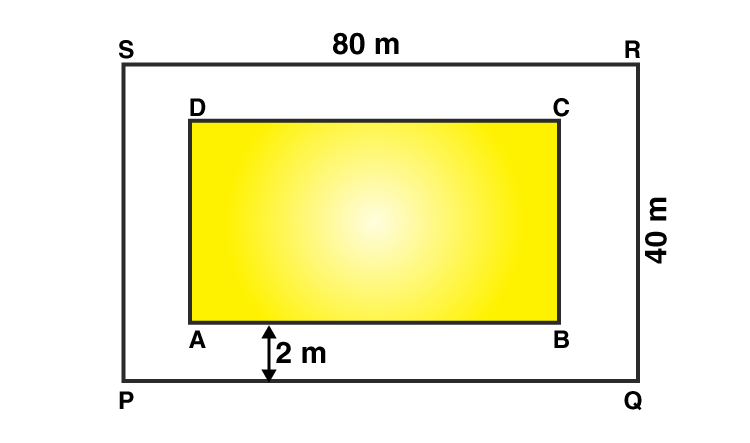Area of the park PQRS = (l × b)

= (80 × 40)

= 3200 m2

Wide of the path = 2 m

Then,

AB = (80 – (2 × 2)) = (80 – 4) = 76 m

AD = (40 – (2 × 2)) = (40 – 4) = 36 m

∴Area of the rectangle ABCD = (76 × 36) m2 = 2736 m2

Area of the path = (Area of PQRS – Area of ABCD)

= (3200 – 2736)

= 464m2

Rate of paving the path = ₹ 80 per m2

∴Total cost of paving the path of a park = ₹ (80 × 464)

= ₹ 37120

8. A school has a hall which is 22 m long and 15.5 m broad. A carpet is laid inside the hall leaving all around a margin of 75 cm from the walls. Find the area of the carpet and the area of the strip left in uncovered. If the width of the carpet is 82 cm, find its cost at the rate of ₹ 60 per m.

Solution:-

Let PQRS be the hall and ABCD be the carpet inside the hall.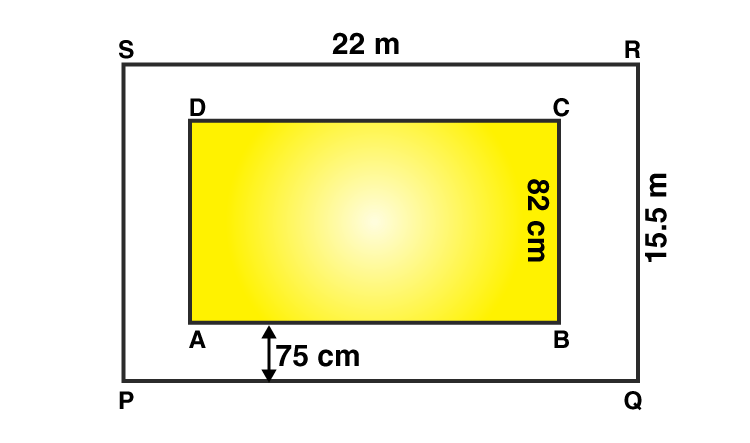School has a hall of length PQ = 22 m

Breadth of the hall PS = 15.5 m

∴Area of the hall PQRS = (l × b) m2

= (22 × 15.5)

= 341 m2

Carpet is laid inside the hall leaving all around a margin of =75 cm = 0.75 m

Then,

The length of the carpet, AB = (22 – (2 × 0.75)) = (22 – (1.5)) = 20.5 m

The breadth of the carpet, AD = (15.5 – (2 × 0.75)) = (15.5 – (1.5)) = 14 m

∴Area of the carpet ABCD = (20.5 × 14) m2 = 287 m2

Area of the Strip = (Area of PQRS – Area of ABCD)

= (341 – 287)

= 54 m2

Now,

Area of 1 m of carpet = 1 × 0.82 = 0.82 m2

∴Length of the carpet whose area 287 m2 = 287/0.82 = 350 m

Hence cost of 350 m long carpet = ₹ (60 × 350)

= ₹ 21000

## Exercise 20c Page: 237

1. Find the area of a parallelogram with base 32 cm and height 16.5 cm.

Solution:-

From the question is given that,

Base of the parallelogram = 32 cm

Height of the parallelogram = 16.5 cm

∴area of the parallelogram = base × height

= 32 × 16.5

= 528 cm2

2. The base of a parallelogram measures 1 m 60 cm and its height is 75 cm. Find its area in m2.

Solution:-

From the question is given that,

Base of the parallelogram = 1 m 60 cm

= 1 m + (60/100) m [Because 1 m = 100 cm]

= 1 + 0.6

= 1.6 m

Height of the parallelogram = 75 cm

= 75/100 [Because 1 m = 100 cm]

= 0.75 m

∴area of the parallelogram = base × height

= 1.6 × 0.75

= 1.2 m2

3. In a parallelogram it is being given that base = 14 dm and height = 6.5 dm. Find its area in (i) cm2

(ii) m2.

Solution:-

(i) Area in cm2

From the question is given that,

Base of the parallelogram = 14 dm

= 14 × 10 [Because 1 dm = 10 cm]

= 140 cm

Height of the parallelogram = 6.5 dm

= 6.5 × 10 [Because 1 dm = 10 cm]

= 65 cm

∴area of the parallelogram = base × height

= 140 × 65

= 9100 cm2

(i) Area in m2

From the question is given that,

Base of the parallelogram = 14 dm

= 14/10 [Because 1 m = 10 dm]

= 1.4 m

Height of the parallelogram = 6.5 dm

= 6.5/10 [Because 1 m = 10 dm]

= 0.65 m

∴area of the parallelogram = base × height

= 1.4 × 0.65

= 0.91 m2

4. Find the height of a parallelogram whose area is 54 cm2 and the base is 15 cm.

Solution:-

From the question is given that,

Base of the parallelogram = 15 cm

Area of the parallelogram = 54 cm2

∴area of the parallelogram = base × height

54 = 15 × height

Height = 54/15

Height = 3.6 cm

Hence, the height of the parallelogram is 3.6 cm

5. One side of a parallelogram is 18 cm long and its area is 153 cm2. Find the distance of the given side from its opposite side.

Solution:-

From the question is given that,

Base of the parallelogram = 18 cm

Area of the parallelogram = 153 cm2

∴area of the parallelogram = base × height

153 = 18 × height

Height = 153/18

Height = 8.5 cm

Hence, the distance of the given side from its opposite side is 8.5 cm

6. In a parallelogram ABCD, AB = 18 cm, BC = 12 cm, AL ⊥ DC and AM ⊥ BC.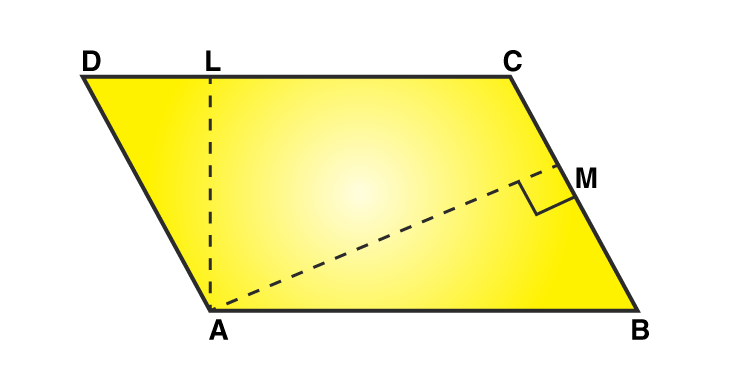If AL = 6.4 cm, find the length of AM.

Solution:-

From the question is given that,

Base of parallelogram, AB = 18 cm

Height of the parallelogram, AL = 6.4 cm

Then,

Area of the parallelogram ABCD = base × height

= 18 × 6.4

= 115.2 cm2 … [eqn. 1]

Now, let us take BC as the base of parallelogram ABCD,

Then,

Area of the parallelogram ABCD = base × height

= 12 × MA

= 12MA cm2 … [eqn. 2]

From the equation (1) and (2)

= 12MA = 115.2 cm2

= MA = (115.2/12)

= MA = 9.6 cm

Hence, the length of AM is 9.6 cm

7. The adjacent sides of a parallelogram are 15 cm and 8 cm. If the distance between the longer sides is 4 cm, find the distance between the shorter sides.

Solution:-

Let us assume ABCD is a parallelogram with side AB of length 15 cm and the corresponding altitude AE of length 4 cm.

The adjacent side AD is of length 8 cm and the corresponding altitude is CF.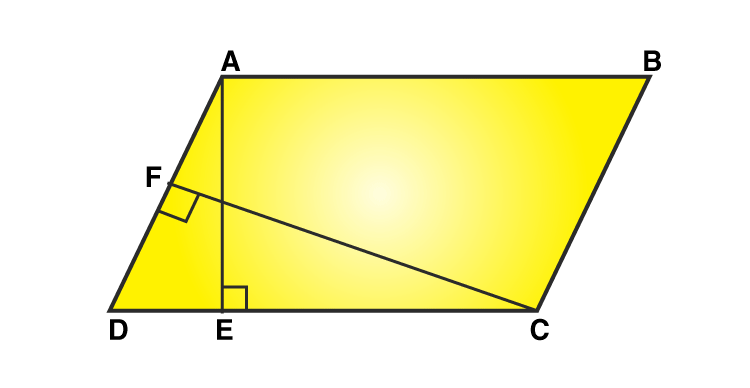WKT, Area of parallelogram = Base × height

Thus, we have two altitudes and two corresponding bases.

∴AD × CF = AB × AE

= 8 cm × CF = 15 cm × 4 cm

= CF = (15 × 4)/8

= CF = (15/2)

= CF = 7.5 cm

∴The distance between the shorter side 7.5 cm

8. The height of a parallelogram is one-third of its base. If the area of the parallelogram is 108 cm2, find its base and height.

Solution:-

Let us assume the base of the parallelogram be x cm.

and height of the parallelogram will be (1/3)x cm.

From the question it is given that the area of the parallelogram is 108 cm2.

∴Area of the parallelogram = base × height

= 108 = (x) × ((1/3)x)

= 108 = (1/3)x2

= x2 = (108 × 3)

= x2 = 324

= x = √324

= x = 18

Then,

The base of the parallelogram be x is 18 cm

Height of the parallelogram will be (1/3)x = (1/3) × 18 = 6 cm

## Exercise 20D Page: 242

1. Find the area of the triangle in which

(i) base = 42 cm and height = 25 cm.

Solution:-

Given,

base = 42 cm

height = 25 cm

∴ Area of the triangle = (1/2) × base × height

= (1/2) × 42 × 25

= 1 × 21 × 25

= 525 cm2

(ii) base = 16.8 m and height = 75 cm.

Solution:-

Convert the given measures into same units.

Given,

base = 16.8 m

height = 75 cm = (75/100) = 0.75 m [because 1 m = 100 cm]

∴ Area of the triangle = (1/2) × base × height

= (1/2) × 16.8 × 0.75

= 0.5 × 16.8 × 0.75

= 6.3 m2

(ii) base = 8 dm and height = 35 cm.

Solution:-

Convert the given measures into same units.

Given,

base = 8 dm = 8 × 10 = 80 cm [because 1 dm = 10 cm]

height = 35 cm

∴ Area of the triangle = (1/2) × base × height

= (1/2) × 80 × 35

= 1 × 40 × 35

= 1400 cm2

2. Find the height of the triangle having an area of 72 cm2 and base 16 cm.

Solution:-

From the question is given that,

Area of the triangle = 72 cm2

Base of the triangle = 16 cm

∴ Area of the triangle = (1/2) × base × height

72 = (1/2) × 16 × height

72 = 1 × 8 × height

Height = 72/8

Height = 9 cm

Hence, the height of the triangle is 9 cm.

3. Find the height of the triangular region having an area of 224 m2 and base 28 m.

Solution:-

From the question is given that,

Area of the triangle = 224 m2

Base of the triangle = 28 m

∴ Area of the triangle = (1/2) × base × height

224 = (1/2) × 28 × height

224 = 1 × 14 × height

Height = 224/14

Height = 16 m

Hence, the height of the triangle is 16 m.

4. Find the base of a triangle whose area is 90 cm2 and height 12 cm.

Solution:-

From the question is given that,

Area of the triangle = 90 cm2

Height of the triangle = 12 cm

∴ Area of the triangle = (1/2) × base × height

90 = (1/2) × base × 12

90 = 1 × 6 × base

Base = 90/6

Base = 15 cm

Hence, the base of the triangle is 15 cm.

5. The base of a triangular field is three times its height. If the cost of cultivating the field at ₹ 1080 per hectare is ₹ 14580, find its base and height.

Solution:-

Total cost of cultivating the field = ₹ 14580

Rate of cultivating the field = ₹ 1080 per hectares

Area of the field = (total cost/rate per hectares)

= (14580/1080)

= 13.5 hectares

= (13.5 × 10000) m2

= 135000 m2

Let the height of the field be x m.

Then, its base = 3x m

Area of the field = (1/2) × (3x) × (x)

= (3x2/2) m2

∴ (3x2/2) = 135000

= x2 = 135000 × (2/3)

= x2 = 90000

= x = √90000

= x = 300

Hence, the height of the field = 300m and its base is 3x = 3 × 300 = 900m

6. The area of the right triangular region is 129.5 cm2. If one of the sides containing the right angle is 14.8 cm, find the other one.

Solution:-

Let the length of the other side be h cm.

Then, area of the triangle = (1/2) × base × height

129.5 = (1/2) × 14.8 × h

129.5 = 1 × 7.4 × h

129.5 = 7.4h

h = 129.5/7.4

h = 17.5 cm

Hence, the length of the other side is 17.5 cm

7. Find the area of a right triangle whose base is 1.2 m and hypotenuse 3.7 m.

Solution:-

Given,

Base = 1.2 m

Hypotenuse = 3. 7 m

Height =?

By Pythagoras theorem, we have

Hypotenuse2 = Base2 + Height2

Height2 = Hypotenuse2 – Base2

Height2 = 3.72 – 1.22

Height2 = 13.69 – 1.44

Height2 = 12.25

Height = √12.25

Height = 3.5 m

Then,

Area of right triangle = (1/2) × base × height

= (1/2) × 1.2 × 3.5

= 0.5 × 1.2 × 3.5

= 2.1 m2

8. The legs of a right triangle are in the ratio 3: 4 and its area is 1014 cm2. Find the lengths of its legs.

Solution:-

Let the legs of the right triangle be 3x and 4x respectively

3x be the base of the right triangle

4x be the height of the right triangle

Then, area of the triangle = (1/2) × base × height

1014 = (1/2) × (3x) × (4x)

1014 = 0.5 × 12x2

1014 = 6x2

X2 = (1014/6)

X2 = 169

X = √169

X = 13

∴The base of the right triangle 3x = 3 × 13 = 39 cm

The height of the right triangle 4x = 4 × 13 = 52 cm

9. One side of a right-angled triangular scarf is 80 cm and its longest side is 1 m. Find its cost at the rate of ₹ 250 per m2.

Solution:-

Let us assume right angle triangular scarf ABC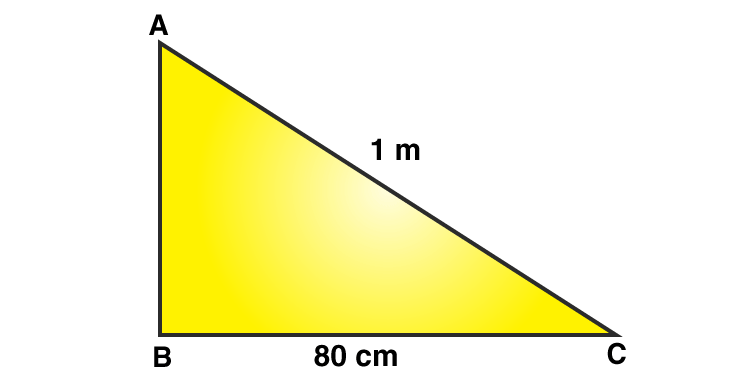Here, ∠B = 90o

BC = 80 cm

AC = 1 m = 100 cm

By Pythagoras theorem, we have

AC2 = BC2 + AB2

AB2 = AC2 – BC2

AB2 = 1002 – 802

AB2 = 10000 – 6400

AB2 = 3600

AB = √3600

AB = 60

Height = 60 cm

Then,

Area of the right-angled triangular scarf = (1/2) × BC × AB

= (1/2) × 80 × 60

= 1 × 40 × 60

= 2400 cm2

= 0.24 [Because 1 m2 = 10000 cm2]

Rate of the cloth ₹ 250 per m2

∴Total cost of the scarf = (250 × 0.24)

= ₹ 60

10. Find the area of an equilateral triangle each of whose sides measures (i) 18 cm, (ii) 20 cm.

Solution:-

(i) 18 cm

Area of the equilateral triangle = ((√3)/4) × (sides)2 sq. Units

= (1.73/4) × (18)2 [because √3 = 1.73]

= (1.73 × 324)/4

= 140.13 cm2

(ii) 20 cm

Area of the equilateral triangle = ((√3)/4) × (sides)2 sq. Units

= (1.73/4) × (20)2 [because √3 = 1.73]

= (1.73 × 400)/4

= 173 cm2

11. The area of an equilateral triangle is (16 × √3) cm2. Find the length of each side of the triangle.

Solution:-

From the question is given that,

Area of an equilateral triangle is (16 × √3) cm2

Area of the equilateral triangle = ((√3)/4) × (sides)2 sq. Units

= (16 × √3) = ((√3)/4) × (sides)2

= (sides)2 = (16 × √3)/ (4/(√3)

= (sides)2 = 16 × 4

= (sides)2 = 64

= Sides = √64

= Sides = 8 cm

Hence, the length of each side of the triangle is 8 cm.

12. Find the length of the height of an equilateral triangle of side 24 cm.

Solution:-

Let us assume the height of an equilateral triangle be h cm.

Then,

Area of the triangle = (1/2) × base × height

= ((1/2) × 24 ×h) cm2

Let the side of the equilateral triangle be a cm

Area of the equilateral triangle = ((√3)/4) × (a)2 sq. Units

= (((√3)/4) × 24 ×24)

= (√3 × 144) cm2

∴ ((1/2) × 24 ×h) = (√3 × 144)

= 12 h = (√3 × 144)

= h = (√3 × 144)/12

= h = (√3 × 12)

= h = 1.73 × 12

= h = 20.76 cm

Hence, the height of an equilateral triangle is 20.76 cm

## Exercise 20E Page: 247

1. Find the circumference of a circle whose radius is

(i) 28 cm, (ii) 1.4 m

Solution:-

(i) 28 cm

Here, radius r = 28 cm

Circumference of a circle = (2πr) units

Where, π = (22/7)

= 2 × (22/7) × 28

= 2 × 22 × 4

= 176 cm

(ii) 1.4 m

Here, radius r = 1.4 m = (14/10) m

Circumference of a circle = (2πr) units

Where, π = (22/7)

= 2 × (22/7) × (14/10)

= 1 × 22 × (2/5)

= 8.8 m

2. Find the circumference of a circle whose diameter is

(i) 35 cm, (ii) 4.9 m

Solution:-

(i) 35 cm

Here, diameter of the circle is given d = 35 cm

Circumference of a circle when diameter is given = πd units

Where, π = (22/7)

= (22/7) × 35

= 22 × 5

= 110 cm

(ii) 4.9 m

Here, diameter of the circle is given d = 4.9 m = (49/10)

Circumference of a circle when diameter is given = πd units

Where, π = (22/7)

= (22/7) × (49/10)

= 11 × (7/5)

= 15.4 m

3. Find the circumference of a circle of radius 15 cm. [Take π = 3.14]

Solution:-

Here, radius r = 15 cm

Circumference of a circle = (2πr) units

Where, π = 3.14 … [given]

= 2 × 3.14 × 15

= 94.2 cm

4. Find the radius of a circle whose circumference is 57.2 cm

Solution:-

Circumference of a given circle is 57.2 cm

∴C = 57.2 cm

Let the radius of the given circle be r cm

Then,

= C = (2πr) units

= r = (C/2π)

= r = (57.2/ (2 × (22/7)))

= r = (57.2/2) × (7/22)

= r = (28.6 × 0.3182)

= r = 9.1 cm

Hence, the radius of a circle is 9.1 cm

5. Find the diameter of a circle whose circumference is 63.8 m

Solution:-

Circumference of a given circle is 63.8 m

∴C = 63.8 m

Let the radius of the given circle be r m

Then,

= C = (2πr) units

= r = (C/2π)

= r = (63.8/ (2 × (22/7)))

= r = (63.8/2) × (7/22)

= r = (31.9 × 0.3182)

= r = 10.15 m

∴Diameter of the circle = 2r = (2 × 10.15) = 20.3 cm

6. The circumference of a circle exceeds its diameter by 30 cm. Find the radius of the circle.

Solution:-

Let the radius of the circle be r cm.

Then, its circumference = (2πr) cm

Now, (circumference) – (diameter) = 30 cm … [given]

∴ (2πr – 2r) = 30

By taking 2r common we get,

= 2r (π – 1) = 30

= 2r × ((22/7) – 1) = 30

= 2r × ((22 – 7)/7) = 30

= 2r × (15/7) = 30

= (30/7) r = 30

= r = (30) × (7/30)

= r = 7 cm

Hence, the radius of the circle is 7 cm.

7. The ratio of the radii of two circle is 5: 3. Find the ratio of their circumferences.

Solution:-

Let the radii of the given circles be 5x and 3x respectively and let their circumferences be C1 and C2 respectively.

Circumference of a circle = 2πr

Then, C1 = 2 × π × 5x = 10πx and C2 = 2 × π × 3x = 6πx

∴ (C1/C2) = (10πx/ 6πx)

= (C1/C2) = (5/ 3)

= (C1: C2) = 5: 3

Hence, the ratio of the circumference of the given circles is 5: 3

8. How long will a man take to make a round of a circular field of radius 21 m, cycling at the speed of 8 km/h?

Solution:-

From the question,

Radius of the circular field, r = 21 m

Cycling at the speed of = 8 km/h

= ((8 × 1000)/ (3600)) m/s … [Because 1 km = 1000 m, 1hr = 3600 sec]

= (8000/3600)

= (80/36) … [divide by 4]

= (20/9) m/s

Distance covered by the cyclist = Circumference of the circular field

= 2πr

= (2 × (22/7) × 21)

= (2 × 22 × 3)

= 132 m

Time taken by the cyclist to cover the field = (Distance covered by the cyclist/Speed of the cyclist)

= (132/ (20/9))

= 132 × (9/20)

= 33 × (9/5)

= 59.4 sec

9. A racetrack is in the form of a ring whose inner circumference is 528 m and the outer circumference is 616 m. Find the width of the track.

Solution:-

Let the inner and outer radii of the track be r meters and R meters respectively.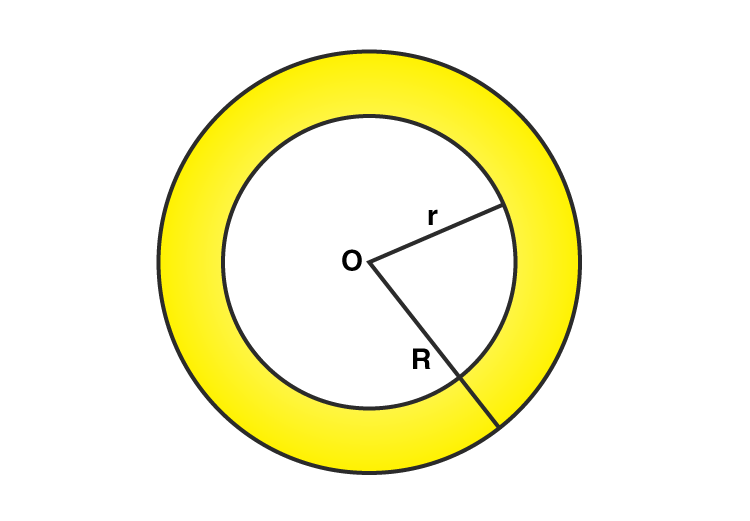Then,

= 2πr = 528 m

= 2 × (22/7) × r = 528

= r = (528/ (2 × (22/7)))

= r = (528/ 2) × (7/22)

= r = (264/2) × (7/11)

= r = 132 × 0.6364

= r = 84 m

And,

= 2πR = 616 m

= 2 × (22/7) × R = 616

= R = (616/ (2 × (22/7)))

= R = (616/ 2) × (7/22)

= R = (308/2) × (7/11)

= R = 154 × 0.6364

= R = 98 m

Now,

(R – r) = (98 – 84)

= 14 m

Hence, the width of the track is 14 m

10. The inner circumference of a circular track is 330 m. The track is 10.5 m wide everywhere. Calculate the cost of putting up a fence along the outer circle at the rate of ₹ 20 per meter.

Solution:-

Let the inner and outer radii of the track be r meters and (r + 10.5) meters respectively.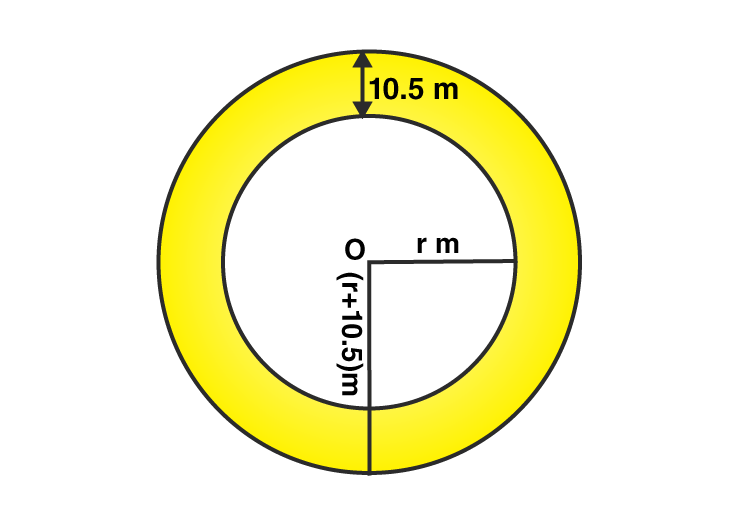Inner circumference = 330 m

∴ 2πr = 330

= 2 × (22/7) × r = 330

= (44/7) × r = 330

= r = 330 × (7/44)

= r = 30 × (7/4)

= r = 52.5 m

Because, Inner radius of the track = 52.5 m

∴Outer radius of the track = (r + 10.5) = (52.5 + 10.5) = 63 m

Then,

Circumference of the outer circle = 2πr

= 2 × (22/7) × 63

= (2 × 22 × 9)

= 396 m

Rate of fencing = ₹ 20 per meter.

Total cost of fencing the outer circle = ₹ (396 × 20)

= ₹ 7920

## Exercise 20F Page: 252

1. Find the area of a circle whose radius is

(i) 21 cm, (ii) 3.5 m

Solution:-

(i) 21 cm

Given, radius r = 21 cm and π = (22/7)

Then,

Area of the circle = πr2 sq units

= (22/7) × 21 ×21

= 22 × 3 × 21

= 1386 cm2

(ii) 3.5 m

Given, radius r = 3.5 m and π = (22/7)

Then,

Area of the circle = πr2 sq units

= (22/7) × 3.5 ×3.5

= 22 × 0.5 × 3.5

= 38.5 m2

2. Find the area of a circle whose diameter is

(i) 28 cm, (ii) 1.4m

Solution:-

(i) 28 cm

Given, Diameter of a circle = 28 cm

WKT, Radius of a circle r = d/2 = 28/2 = 14 cm

Then,

Area of the circle = πr2 sq units

= (22/7) × 14 ×14

= 22 × 2 × 14

= 616 cm2

(ii) 1.4 m

Given, Diameter of a circle = 1.4 m

WKT, Radius of a circle r = d/2 = 1.4/2 = 0.7 m

Then,

Area of the circle = πr2 sq units

= (22/7) × 0.7 × 0.7

= 22 × 0.1 × 0.7

= 1.54 m2

3. The circumference of a circle is 264 cm. Find its area.

Solution:-

Let the radius of the circle be r cm.

Then, its circumference = 2πr cm

∴ 2πr = 264

= 2 × (22/7) × r = 264

= (44/7) × r = 264

= r = 264 × (7/44)

= r = 42 cm

∴Area of the circle = πr2

= (22/7) × 42 × 42

= 22 × 6 × 42

= 5544 cm2

4. The circumference of a circle is 35.2 m. Find its area.

Solution:-

Let the radius of the circle be r m.

Then, its circumference = 2πr m

∴ 2πr = 35.2

= 2 × (22/7) × r = 35.2

= (44/7) × r = 35.2

= r = 35.2 × (7/44)

= r = 5.6 m

∴Area of the circle = πr2

= (22/7) × 5.6 × 5.6

= 22 × 0.8 × 5.6

= 98.56 m2

5. The area of a circle is 616 cm2. Find its circumference.

Solution:-

Let the radius of a circle be r cm.

Then, its area = πr2 cm2

= 616 = (22/7) × r2

= r2 = 616 × (7/22)

= r2 = 308 × (7/11)

= r = √ (196)

= r = 14 cm

Now,

Circumference of a circle = 2 πr

= 2 × (22/7) × 14

= 2 × 22 × 2

= 88 cm

6. The area of a circle is 1386 m2. Find its circumference.

Solution:-

Let the radius of a circle be r meters.

Then, its area = πr2 m2

= 1386 = (22/7) × r2

= r2 = 1386 × (7/22)

= r2 = 693 × (7/11)

= r = √(441)

= r = 21 m

Now,

Circumference of a circle = 2 πr

= 2 × (22/7) × 21

= 2 × 22 × 3

= 132 m

7. The ratio of the radii of two circles is 4: 5. Find the ratio of their areas.

Solution:-

Let the radii of the given circles be 4x and 5x respectively and let their areas be A1 and A2 respectively.

Then, A1 = πr2

= A1 = π × (4x)2

= A1 = 16πx2

And A2 = πr2

= A2 = π × (5x)2

= A2 = 25πx2

∴ (A1/A2) = (16πx2)/ (25πx2)

(A1/A2) = (16/25)

A1: A2 = 16: 25

Hence, the ratio of the areas of two circles is 16: 25

8. A horse is tied to a pole in a park with a string 21 m long. Find the area over which the horse can graze.

Solution:-

From the question,

Horse is tied to a pole, then the pole will be the central point and the area over which the horse will graze will be a circle. The string by which the horse is tied will be the radius of the circle.

Then,

Radius of the circle, r = length of the string = 21 m

Then, area of the circle = πr2

= (22/7) × 21 × 21

= 22 × 3 × 21

= 1386 m2

Hence, the area of the horse can graze is 1386 m2.

## Exercise 20G Page: 253

Mark against the correct answer in each of the following:

1. The length of a rectangle is 16 cm and the length of its diagonal is 20 cm. The area of the rectangle is

(a) 320 cm2 (b) 160 cm2 (c) 192 cm2 (d) 156 cm2

Solution:-

(c) 192 cm2

Because,

Let ABCD be the rectangular plot.

Then, AB = 16 cm and AC = 20 cm

BC =?

According to Pythagoras theorem,

From right angle triangle ABC, we have:

= AC2 = AB2 + BC2

= 202 = 162 + BC2

= BC2 = 202 – 162

= BC2 = 400 – 256

= BC2 = 144

= BC = √144

= BC = 12 cm

Hence, the area of the rectangle plot = (l × b)

Where, l = 16 cm, b = 12 cm

Then,

= (16 × 12)

= 192 cm2

2. Each diagonal of a square is 12 cm long. Its area is

(a) 144 cm2 (b) 72 cm2 (c) 36 cm2 (d) none of these

Solution:-

(b) 72 cm2

Because,

Given,

Diagonal of a square = 12 cm

Area of a square = (1/2) × (diagonal)2

= (1/2) × 12 × 12

= 1 × 6 × 12

= 72cm2

3. The area of a square is 200 cm2. The length of its diagonal is

(a) 10 cm (b) 20 cm (c) 10√2 cm (d) 14.1 cm

Solution:-

(b) 20 cm

Because,

We know that,

Area of a square = (1/2) × (diagonal)2

200 = (1/2) × (diagonal)2

(Diagonal)2 = 200 × (2/1)

Diagonal = √400

Diagonal = 20 cm

4. The area of a square field is 0.5 hectare. The length of its diagonal is

(a) 100 m (b) 50 m (c) 250 m (d) 50√2 m

Solution:-

(a) 100 m

Because,

1 hectare = 10000 m2

∴ Area of square field = 0.5 × 10000 = 5000 m2

WKT, Area of a square = (1/2) × (diagonal)2

5000 = (1/2) × (diagonal)2

(Diagonal)2 = 5000 × (2/1)

Diagonal = √10000

Diagonal = 100 m

5. The length of a rectangular field is thrice its breadth and its perimeter is 240 m. The length of the field is

(a) 80 m (b) 120 m (c) 90 m (d) none of these

Solution:-

(c) 90 m

Because,

Let us assume the Breadth of the rectangular field be x m.

Let us assume the length of the rectangular field is thrice its breadth be 3x m

Then,

Perimeter of the rectangular field = 2(l + b)

= 240 = 2(x + 3x)

= 240 = 2(4x)

= 240 = 8x

= x = 240/8

= x = 30

∴the length of the rectangular field is 3x = 3 × 30 = 90 m

6. On increasing each side of a square by 25 %, the increase in area will be

(a) 25% (b) 55% (c) 40.5% (d) 56.25%

Solution:-

(d) 56.25%

Because,

Let the length of each side be a cm

Then, its area = a2 cm2

Increased side = (a + 25% 0of a) cm

= (a + (1/4) a)

= (5/4)a cm

Area of the square = ((5/4)a)2

= (25/16)a2 cm2

Increase in area = [(25/16)a2 – a2] cm

= (25a2 – 16a2)/ (16)

= (9a2/16) cm2

% increase in the area = (Increased area / old area) × 100

= [(9a2/16)/ a2] × 100

= (9 × 100)/ 16

= 56.25%

7. The area of a square and that of a square drawn on its diagonal are in the ratio

(a) 1: √2 (b) 1: 2 (c) 1: 3 (d) 1: 4

Solution:-

(b) 1: 2

Because,

Let the side of square be a.

And Length of its diagonal = √2 a

∴Required ratio = a2/ (√2a)2

= a2/2a2

= ½

= 1: 2

8. The perimeters of a square and a rectangle are equal. If their areas be A m2 and B m2, then which of the following is a true statement?

(a) A < B (b) A < B (c) A > B (d) A > B

Solution:-

(c) A > B

Because,

(area of the square) > (area of the rectangle), when perimeters are equal.

9. The length and breadth of a rectangular field are in the ratio 5: 3 and its perimeter is 480 m. The area of the field is

(a) 7200 m2 (b) 13500 m2 (c) 15000 m2 (d) 540000 m2

Solution:-

(b) 13500 m2

Because,

Let the length of the rectangular field = 5x

The breadth of the rectangular field = 3x

Then,

perimeter of the rectangular land = 2 ( l + b )

480= 2 (5x + 3x)

480 = 2 (8x)

480 = 16x

x = (480/16)

x = 30

∴ the length of the rectangular field = 5x = 5 × 30 = 150m

The breadth of the rectangular field = 3x = 3 × 30 = 90m

Area of rectangular field = (l × b) sq units

Then,

= (150 × 90)

= 13500 m2

10. The length of a room is 15 m. the cost of carpeting it with a carpet 75 cm wide at ₹ 50 per meter is ₹ 6000. The width of the room is

(a) 6 m (b) 8 m (c) 13.4 m (d) 18 m

Solution:-

(a) 6 m

Because,

From the question,

Total cost of carpeting = ₹ 6000

Rate of carpeting = ₹ 50 per meter

Then,

Length of the carpet = (6000/50) = 120 m

∴Area of the carpet = (120 × (75/100))

= 90 m2

Area of the floor = Area of the carpet = 90 m2

Hence, the width of the room = (Area/length)

= (90/15)

= 6 m

11. The sides of a triangle measures 13 cm, 14 cm and 15 cm. Its area is

(a) 84 cm2 (b) 91 cm2 (c) 168 cm2 (d) 182 cm2

Solution:-

(a) 84 cm2

Because,

Let a, b, c be the sides of the triangle

Then, s = (1/2) (a + b + c)

= (1/2) × (13 + 14 + 15)

= (42/2)

= 21

Area of triangle = √(s(s-a) × (s-b) × (s-c))

= √(21 × (21 – 13) × (21 – 14) × (21 – 15))

= √(21 × (8) × (7) × (6))

= √(3 × 7 × 2 × 2 × 2 × 7 × 2 × 3)

= √(32 × 72 × 22 × 22)

By cancelling square and square root we get,

= (3 × 7 × 2 × 2)

= 84 cm2

12. The base and height of a triangle is are 12 m and 8 m respectively. Its area is

(a) 96 m2 (b) 48 m2 (c) 16√3 m2 (d) 16√2 m2

Solution:-

(b) 48 m2

Because,

Given,

base = 12 m

height = 8 m

∴ Area of the triangle = (1/2) × base × height

= (1/2) × 12 × 8

= 1 × 6 × 8

= 48 m2

13. The area of an equilateral triangle is 4√3 cm2. The length of each of its sides is

(a) 3 cm (b) 4 cm (b) 2√3 cm (d) ½ √3 cm

Solution:-

(b) 4 cm

Because,

From the question is given that,

Area of an equilateral triangle is (4√3) cm2

Area of the equilateral triangle = ((√3)/4) × (sides)2 sq. Units

= (4√3) = ((√3)/4) × (sides)2

= (sides)2 = (4√3)/ (4/(√3)

= (sides)2 = (4√3 × 4)/ √3

= (sides)2 = 4 × 4

= Sides = √16

= Sides = 4 cm

Hence, the length of each side of the triangle is 4 cm.

14. Each side of an equilateral triangle is 8 cm long. Its area is

(a) 32 cm2 (b) 64 cm2 (c) 16√3 cm2 (d) 16√2 cm2

Solution:-

(c) 16√3 cm2

Because,

Area of the equilateral triangle = ((√3)/4) × (sides)2 sq. Units

= (√3 /4) × (8)2

= (√3/4) × 64)

= (√3 × 16)

= 16√3 cm2

15. The height of an equilateral triangle is √6 cm. Its area is

(a) 3√3 cm2 (b) 2√3 cm2 (c) 2√2 cm2 (d) 6√2 cm2

Solution:-

(b) 2√3 cm2

Because,

Let an equilateral triangle with one side of length a cm

Diagonal of an equilateral triangle = (√3/2) a

= (√3/2) a = √6

= a = (√6 × 2)/ √3

= a = (√3 × √2 × 2)/ √3

= a = 2√2

Area of equilateral triangle = √3/4 a2

= √3/4 × (2√2)2

= (√3/4) × 8

= 2√3 cm2

16. One side of a parallelogram is 16 cm and the distance of this side from the opposite side is 4.5 cm. The area of the parallelogram is

(a) 36 cm2 (b) 72 cm2 (c) 18 cm2 (d) 54 cm2

Solution:-

(b) 72 cm2

Because,

From the question is given that,

Base of the parallelogram = 16 cm

Height of the parallelogram = 4.5 cm

∴area of the parallelogram = base × height

= 16 × 4.5

= 72 cm2

## RS Aggarwal Solutions for Class 7 Maths Chapter 20 – Mensuration

Chapter 20 – Mensuration contains 7 exercises and the RS Aggarwal Solutions available on this page provide solutions to the questions present in the exercises. Now, let us have a look at some of the concepts discussed in this chapter.

• Area of Rectilinear Figures
• Standard Units of Area
• Area and Perimeter of a Rectangle
• Area and Perimeter of a Square
• Area Between Two Rectangles
• Areas of Parallelogram and Rhombus
• Areas of Triangles
• Circumference and Area of a Circle

### Chapter Brief of RS Aggarwal Solutions for Class 7 Maths Chapter 20 – Mensuration

RS Aggarwal Solutions for Class 7 Maths Chapter 20 – Mensuration is a topic in Geometry which is a branch of Mathematics. Mensuration deals with length, area and volume of different kinds of shapes both 2D and 3D. Mensuration is a formula oriented topic. There are specific formulas for various parameters; we put the values in these Mensuration formulas and get the desired answers. But at the same time, it is also a calculation heavy topic, so we suggest you use smart methods for these calculations.